https://doi.org/10.5194/gmd-13-955-2020
https://doi.org/10.5194/gmd-13-955-2020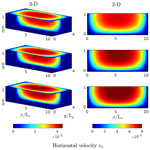# Modelling thermomechanical ice deformation using an implicit pseudo-transient method (FastICE v1.0) based on graphical processing units (GPUs)

Ludovic Räss, Aleksandar Licul, Frédéric Herman, Yury Y. Podladchikov, and Jenny Suckale
Abstract

Ice sheets lose the majority of their mass through outlet glaciers or ice streams, corridors of fast ice moving multiple orders of magnitude more rapidly than the surrounding ice. The future stability of these corridors of fast-moving ice depends sensitively on the behaviour of their boundaries, namely shear margins, grounding zones and the basal sliding interface, where the stress field is complex and fundamentally three-dimensional. These boundaries are prone to thermomechanical localisation, which can be captured numerically only with high temporal and spatial resolution. Thus, better understanding the coupled physical processes that govern the response of these boundaries to climate change necessitates a non-linear, full Stokes model that affords high resolution and scales well in three dimensions. This paper's goal is to contribute to the growing toolbox for modelling thermomechanical deformation in ice by leveraging graphical processing unit (GPU) accelerators' parallel scalability. We propose FastICE, a numerical model that relies on pseudo-transient iterations to solve the implicit thermomechanical coupling between ice motion and temperature involving shear heating and a temperature-dependent ice viscosity. FastICE is based on the finite-difference discretisation, and we implement the pseudo-time integration in a matrix-free way. We benchmark the mechanical Stokes solver against the finite-element code Elmer/Ice and report good agreement among the results. We showcase a parallel version of FastICE to run on GPU-accelerated distributed memory machines, reaching a parallel efficiency of 99 %. We show that our model is particularly useful for improving our process-based understanding of flow localisation in the complex transition zones bounding rapidly moving ice.

Share
Dates
1 Introduction

The fourth IPCC report concludes that existing ice-sheet flow models do not accurately describe polar ice-sheet discharge owing to their inability to simultaneously model slow and fast ice motion . This issue results from the fact that many ice-flow models are based on simplified approximations of non-linear Stokes equations, such as first-order Stokes , shallow shelf and shallow ice models. Shallow ice models are computationally more tractable and describe the motion of large homogeneous portions of ice as a function of the basal friction. However, this category of models fails to capture the coupled multiscale processes that govern the behaviour of the boundaries of streaming ice, including shear margins, grounding zones and the basal interface. These boundaries dictate the stability of the current main drainage routes from Antarctica and Greenland, and predicting their future evolution is critical for understanding polar ice-sheet discharge.

Full Stokes models provide a complete mechanical description of deformation by capturing the entire stress rate and strain rate tensor. In three dimensions (3-D), full Stokes calculations set a high demand on computational resources that requires a parallel and high-performance computing approach to achieve reasonable times to solution. An added challenge in full Stokes models is the strongly non-linear thermomechanics of ice. Ice viscosity significantly depends on both temperature and strain rate , which can lead to spontaneous localisation of shear (e.g. Duretz et al.2019; Räss et al.2019a). Particularly challenging is the scale separation associated with localisation, which leads to microscale physical interaction generating mesoscale features such as thermally activated shear zones or preferential flow paths in macroscale ice domains. Thus, both high spatial and temporal resolutions are important for numerical models to capture and resolve spontaneous localisation.

The main contribution of this paper is to leverage the unprecedented parallel performance of modern graphical processing units (GPUs) to accelerate the time to solution for thermomechanically coupled full Stokes models in 3-D utilising a pseudo-transient (PT) iterative scheme – FastICE . FastICE is a process-based model that focuses specifically on improving our ability to better model and understand spontaneous englacial instabilities such as thermomechanical localisation at the scale of individual field sites. Thermomechanical localisation arises in a self-consistent way in shear margins, at the grounding zone and in the vicinity of the basal sliding interface, making our model particularly well suited for assessing the complex physical feedbacks in the boundaries of fast-moving ice. FastICE is a complement to existing models by providing a multi-physics platform for studying the transition between fast and slow ice motion rather than addressing the large-scale evolution of the entire ice sheet.

Recent trends in the computing industry show a shift from single-core to many-core architectures as an effective way to increase computational performance. This trend is common to both central processing unit (CPU) and GPU hardware architectures (Cook2012). GPUs are compact, affordable and relatively programmable devices that offer high-performance throughput (close to TB per second peak memory throughput) and a good price-to-performance ratio. GPUs offer an attractive alternative to conventional CPUs owing to their massively parallel architecture featuring thousands of cores. The programming model behind GPUs is based on a parallel principle called single instruction multiple data (SIMD). This principle entails every single instruction being executed on different data. The same instruction block is executed by every thread. GPUs' massive parallelism and the related high performance is achieved by executing thousands of threads concurrently using multi-threading in order to effectively hide latency. Numerical stencil-based techniques such as the finite-difference method allow one to take advantage of GPU hardware, since spatial derivatives are approximated by differences between two (or more) adjacent grid points. This results in minimal, local and regular memory access patterns. The operations performed on each stencil are identical for each grid point throughout the entire computational domain. Combined with a matrix-free discretisation of the equations and iterative PT updates, the finite-difference stencil evaluation is well suited for the SIMD programming philosophy of GPUs. Each operation on the GPU assigns one thread to compute the update of a given grid point. Since on the GPU device, one core can simultaneously execute several threads, the operation set is executed on the entire computational domain almost concurrently.

We tailor our numerical method to optimally exploit the massive parallelism of GPU hardware, taking inspiration from recent successful GPU-based implementations of viscous and coupled flow problems . Our work is most comparable to the few land–ice dynamical cores targeting many-core architectures such as GPUs . Our numerical implementation relies on an iterative and matrix-free method to solve the mechanical and thermal problems using a finite-difference discretisation on a Cartesian staggered grid. We ensure optimal performance, minimising the memory footprint bottleneck while ensuring optimal data alignment in computer memory. Our accelerated PT algorithm utilises an analogy of transient physics to converge to the steady-state problem at every time step. One advantage of this approach is that the iterative stability criterion is physically motivated and intuitive to adjust and to generalise. Using transient physics for numerical purposes allows us to define local CFL-like (Courant–Friedrich–Lewy) criteria in each computational cell to be used to minimise residuals. This approach enables a maximal convergence rate simultaneously in the entire domain and avoids costly global reduction operations from becoming a bottleneck in parallel computing.

We verify the numerical implementation of our mechanical Stokes solver against available benchmark studies including EISMINT and ISMIP . There is only one model inter-comparison that investigates the coupled thermomechanical dynamics, EISMINT 2 . Unfortunately, experiments in EISMINT 2 are usually performed using a coupled thermomechanical first-order shallow ice model , making the comparison to our full Stokes implementation less immediate. Although thermomechanically coupled Stokes models exist , very few studies have investigated key aspects of the implemented model, such as convergence among grid refinement and impacts of one-way vs. two-way couplings, with few exceptions (e.g. Duretz et al.2019).

We start by providing an overview of the mathematical model, describing ice dynamics and its numerical implementation. We then discuss GPU capabilities and explain our GPU implementation. We further report model comparison against a selection of benchmark studies, followed by sharing the results and performance measurements. Finally, we discuss pros and cons of the method and highlight glaciological contexts in which our model could prove useful. The code examples based on the PT method in both the MATLAB and CUDA C programming language are available for download from Bitbucket at: https://bitbucket.org/lraess/fastice/ (last access: 2 March 2020) and from: http://wp.unil.ch/geocomputing/software/ (last access: 2 March 2020).

2 The model

## 2.1 The mathematical model

We capture the flow of an incompressible, non-linear, viscous fluid – including a temperature-dependent rheology. Since ice is approximately incompressible, the equation for conservation of mass reduces to

$\begin{array}{}\text{(1)}& \frac{\partial {v}_{i}}{\partial {x}_{i}}=\mathrm{0}\phantom{\rule{0.25em}{0ex}},\end{array}$

where vi is the velocity component in the spatial direction xi.

Neglecting inertial forces, ice's flow is driven by gravity and is resisted by internal deformation and basal stress:

$\begin{array}{}\text{(2)}& \frac{\partial {\mathit{\tau }}_{ij}}{\partial {x}_{j}}-\frac{\partial P}{\partial {x}_{i}}+{F}_{i}=\mathrm{0}\phantom{\rule{0.25em}{0ex}},\end{array}$

where ${F}_{i}=\mathit{\rho }g\mathrm{sin}\left(\mathit{\alpha }\right)\left[\mathrm{1},\mathrm{0},-\mathrm{cot}\left(\mathit{\alpha }\right)\right]$ is the external force. Ice density is denoted by ρ, g is the gravitational acceleration and α is the characteristic bed slope. P is the isotropic pressure and τij is the deviatoric stress tensor. The deviatoric stress tensor τij is obtained by decomposing the Cauchy stress tensor σij in terms of deviatoric stress τij and isotropic pressure P.

In the absence of phase transitions, the temporal evolution of temperature in deforming, incompressible ice is governed by advection, diffusion and shear heating:

$\begin{array}{}\text{(3)}& \mathit{\rho }c\left(\frac{\partial T}{\partial t}+{v}_{i}\frac{\partial T}{\partial {x}_{i}}\right)=\frac{\partial }{\partial {x}_{i}}\left(k\frac{\partial T}{\partial {x}_{i}}\right)+{\mathit{\tau }}_{ij}{\stackrel{\mathrm{˙}}{\mathit{ϵ}}}_{ij}\phantom{\rule{0.25em}{0ex}},\end{array}$

where T represents the temperature deviation from the initial temperature T0, c is the specific heat capacity, k is the spatially varying thermal conductivity and ${\stackrel{\mathrm{˙}}{\mathit{ϵ}}}_{ij}$ is the strain rate tensor. The term ${\mathit{\tau }}_{ij}{\stackrel{\mathrm{˙}}{\mathit{ϵ}}}_{ij}$ represents the shear heating, a source term that emerges from the mechanical model.

Shear heating could locally raise the temperature in the ice to the pressure melting point. Once ice has reached the melting point, any additional heating is converted to latent heat, which prevents further temperature increase. Thus, we impose a temperature cap at the pressure melting point, following , by describing the melt production using a Heaviside function χ(TTm):

$\begin{array}{}\text{(4)}& \mathit{\rho }c\left(\frac{\partial T}{\partial t}+{v}_{i}\frac{\partial T}{\partial {x}_{i}}\right)=\frac{\partial }{\partial {x}_{i}}\left(k\frac{\partial T}{\partial {x}_{i}}\right)+\left[\mathrm{1}-\mathit{\chi }\left(T-{T}_{\mathrm{m}}\right)\right]{\mathit{\tau }}_{ij}{\stackrel{\mathrm{˙}}{\mathit{ϵ}}}_{ij}\phantom{\rule{0.25em}{0ex}},\end{array}$

where Tm stands for the ice melting temperature. We balance the heat produced by shear heating with a sink term in regions where the melting temperature is reached. The volume of produced meltwater can be calculated in a similar way as proposed by .

We approximate the rheology of ice through Glen's flow law (Glen1952; Nye1953):

$\begin{array}{}\text{(5)}& {\stackrel{\mathrm{˙}}{\mathit{ϵ}}}_{ij}=\frac{\mathrm{1}}{\mathrm{2}}\left(\frac{\partial {v}_{i}}{\partial {x}_{j}}+\frac{\partial {v}_{j}}{\partial {x}_{i}}\right)={a}_{\mathrm{0}}{{\mathit{\tau }}_{\mathrm{II}}}^{n-\mathrm{1}}\mathrm{exp}\left(-\frac{Q}{R\left(T+{T}_{\mathrm{0}}\right)}\right){\mathit{\tau }}_{ij}\phantom{\rule{0.25em}{0ex}},\end{array}$

where a0 is the pre-exponential factor, R is the universal gas constant, Q is the activation energy, n is the stress exponent and τII is the second invariant of the stress tensor defined by ${\mathit{\tau }}_{\mathrm{II}}=\sqrt{\mathrm{1}/\mathrm{2}{\mathit{\tau }}_{ij}{\mathit{\tau }}_{ij}}$. Glen's flow law posits an exponent of n=3.

At the ice-top surface Γt(t), we impose the upper surface boundary condition ${\mathit{\sigma }}_{ij}\phantom{\rule{0.25em}{0ex}}{n}_{j}=-{P}_{\mathrm{atm}}{n}_{j}$, where nj denotes the normal unit vector at the ice surface boundary and Patm the atmospheric pressure. Because atmospheric pressure is negligible relative to pressure within the ice column, we can also use a standard stress-free simplification of the upper surface boundary condition σijnj=0. On the bottom ice–bedrock interface, we can impose two different boundary conditions. For the parts of the ice–bedrock interface Γ0(t) where the ice is frozen to the ground, we impose a zero velocity vi=0 and thus no sliding boundary condition. On the parts of the ice–bedrock interface Γs(t) where the ice is at the melting point, we impose a Rayleigh friction boundary condition – the so-called linear sliding law – given by

$\begin{array}{}\text{(6)}& \begin{array}{rl}& {v}_{i}{n}_{\mathrm{i}}=\mathrm{0}\phantom{\rule{0.25em}{0ex}},\\ & {n}_{\mathrm{i}}{\mathit{\sigma }}_{ij}{t}_{j}=-{\mathit{\beta }}^{\mathrm{2}}{v}_{j}{t}_{j}\phantom{\rule{0.25em}{0ex}},\end{array}\end{array}$

where the parameter β2 denotes a given sliding coefficient, ni denotes the normal unit vector at the ice–bedrock interface and tj denotes any unit vector tangential to the bottom surface. On the side or lateral boundaries, we impose either Dirichlet boundary conditions if the velocities are known or periodic boundary conditions, mimicking an infinitely extended domain.

## 2.2 Non-dimensionalisation

For numerical purposes and for ease of generalisation, it is often preferable to use non-dimensional variables. This allows one to limit truncation errors (especially relevant for single-precision calculations) and to scale the results to various different initial configurations. Here, we use two different scale sets, depending on whether we solve the purely mechanical part of the model or the thermomechanically coupled system of equations.

In the case of an isothermal model, we use ice thickness, H, and gravitational driving stress to non-dimensionalise the governing equations:

$\begin{array}{}\text{(7)}& \begin{array}{rl}\stackrel{\mathrm{‾}}{L}& =H\phantom{\rule{0.25em}{0ex}},\\ \stackrel{\mathrm{‾}}{\mathit{\tau }}& =\mathit{\rho }g\stackrel{\mathrm{‾}}{L}\mathrm{sin}\left(\mathit{\alpha }\right),\phantom{\rule{0.25em}{0ex}},\\ \stackrel{\mathrm{‾}}{v}& ={\mathrm{2}}^{n}{A}_{\mathrm{0}}\stackrel{\mathrm{‾}}{L}{\stackrel{\mathrm{‾}}{\mathit{\tau }}}^{n}\phantom{\rule{0.25em}{0ex}},\end{array}\end{array}$

where A0 is the isothermal deformation rate factor and α is the mean bed slope. We can then rewrite the governing equations in their non-dimensional form as follows:

$\begin{array}{}\text{(8)}& \begin{array}{rl}& \frac{\partial {v}_{i}^{\prime }}{\partial {x}_{i}^{\prime }}=\mathrm{0}\phantom{\rule{0.25em}{0ex}},\\ & \frac{\partial {\mathit{\tau }}_{ij}^{\prime }}{\partial {x}_{j}^{\prime }}-\frac{\partial {P}^{\prime }}{\partial {x}_{i}^{\prime }}+{F}_{i}^{\prime }=\mathrm{0}\phantom{\rule{0.25em}{0ex}},\\ & {\stackrel{\mathrm{˙}}{{\mathit{ϵ}}^{\prime }}}_{ij}=\frac{\mathrm{1}}{\mathrm{2}}\left(\frac{\partial {v}_{i}^{\prime }}{\partial {x}_{j}^{\prime }}+\frac{\partial {v}_{j}^{\prime }}{\partial {x}_{i}^{\prime }}\right)={\mathrm{2}}^{-n}{{\mathit{\tau }}_{\mathrm{II}}^{\prime }}^{n-\mathrm{1}}{\mathit{\tau }}_{ij}^{\prime }\phantom{\rule{0.25em}{0ex}},\end{array}\end{array}$

where ${F}_{i}^{\prime }$ is now defined as ${F}_{i}^{\prime }=\left[\mathrm{1},\mathrm{0},-\mathrm{cot}\left(\mathit{\alpha }\right)\right]$. The model parameters are the mean bed slope α and domain size in each horizontal direction, i.e. ${L}_{x}^{\prime }$ and ${L}_{y}^{\prime }$.

Reducing the thermomechanically coupled equations to a non-dimensional form requires not only length and stress, but also temperature and time. We choose the characteristic scales such that the coefficients in front of the diffusion and shear heating terms in the temperature evolution Eq. (3) reduce to 1:

$\begin{array}{}\text{(9)}& \begin{array}{rl}\stackrel{\mathrm{‾}}{T}& =\frac{nR{{T}_{\mathrm{0}}}^{\mathrm{2}}}{Q}\phantom{\rule{0.25em}{0ex}},\\ \stackrel{\mathrm{‾}}{\mathit{\tau }}& =\mathit{\rho }{c}_{\mathrm{p}}\stackrel{\mathrm{‾}}{T}\phantom{\rule{0.25em}{0ex}},\\ \stackrel{\mathrm{‾}}{t}& ={\mathrm{2}}^{-n}{a}_{\mathrm{0}}^{-\mathrm{1}}{\stackrel{\mathrm{‾}}{\mathit{\tau }}}^{-n}\mathrm{exp}\left(\frac{Q}{R{T}_{\mathrm{0}}}\right)\phantom{\rule{0.25em}{0ex}},\\ \stackrel{\mathrm{‾}}{L}& =\sqrt{\frac{k}{\mathit{\rho }{c}_{\mathrm{p}}}\stackrel{\mathrm{‾}}{t}}\phantom{\rule{0.25em}{0ex}}.\end{array}\end{array}$

These choices entail the velocity scale in the thermomechanical model being $\stackrel{\mathrm{‾}}{v}=\stackrel{\mathrm{‾}}{L}/\stackrel{\mathrm{‾}}{t}$. We obtain the non-dimensional (primed variables) by using the characteristic scales given in Eq. (9), which leads to

$\begin{array}{}\text{(10)}& \begin{array}{rl}& \frac{\partial {v}_{i}^{\prime }}{\partial {x}_{i}^{\prime }}=\mathrm{0}\phantom{\rule{0.25em}{0ex}},\\ & \frac{\partial {\mathit{\tau }}_{ij}^{\prime }}{\partial {x}_{j}^{\prime }}-\frac{\partial {P}^{\prime }}{\partial {x}_{i}^{\prime }}+{F}_{i}^{\prime }=\mathrm{0}\phantom{\rule{0.25em}{0ex}},\\ & \frac{\partial {T}^{\prime }}{\partial {t}^{\prime }}+{v}_{i}^{\prime }\frac{\partial {T}^{\prime }}{\partial {x}_{i}^{\prime }}=\frac{{\partial }^{\mathrm{2}}{T}^{\prime }}{\partial {{x}_{i}^{\prime }}^{\mathrm{2}}}+{\mathit{\tau }}_{ij}^{\prime }{\stackrel{\mathrm{˙}}{{\mathit{ϵ}}^{\prime }}}_{ij}\phantom{\rule{0.25em}{0ex}},\\ & {\stackrel{\mathrm{˙}}{{\mathit{ϵ}}^{\prime }}}_{ij}=\frac{\mathrm{1}}{\mathrm{2}}\left(\frac{\partial {v}_{i}^{\prime }}{\partial {x}_{j}^{\prime }}+\frac{\partial {v}_{j}^{\prime }}{\partial {x}_{i}^{\prime }}\right)\\ & \phantom{\rule{0.25em}{0ex}}\phantom{\rule{0.25em}{0ex}}\phantom{\rule{1em}{0ex}}={\mathrm{2}}^{-n}{{\mathit{\tau }}_{\mathrm{II}}^{\prime }}^{n-\mathrm{1}}\mathrm{exp}\left(\frac{n{T}^{\prime }}{\mathrm{1}+\frac{{T}^{\prime }}{{T}_{\mathrm{0}}^{\prime }}}\right){\mathit{\tau }}_{ij}^{\prime }\phantom{\rule{0.25em}{0ex}},\end{array}\end{array}$

where ${F}_{i}^{\prime }$ is now defined as ${F}_{i}^{\prime }=\stackrel{\mathrm{‾}}{F}\left[\mathrm{1},\mathrm{0},-\mathrm{cot}\left(\mathit{\alpha }\right)\right]$ and $\stackrel{\mathrm{‾}}{F}=\mathit{\rho }g\mathrm{sin}\left(\mathit{\alpha }\right)\stackrel{\mathrm{‾}}{L}/\stackrel{\mathrm{‾}}{\mathit{\tau }}$. The model parameters are the non-dimensional initial temperature ${T}_{\mathrm{0}}^{\prime }$, the stress exponent n, the non-dimensional force $\stackrel{\mathrm{‾}}{F}$, the mean bed slope α, non-dimensional domain height ${L}_{z}^{\prime }$, and the horizontal domain size ${L}_{x}^{\prime }$ and ${L}_{y}^{\prime }$ (Fig. 3). We motivate the chosen characteristic scales by their usage in other studies of thermomechanical strain localisation . In the interest of a simple notation, we will omit the prime symbols on all non-dimensional variables in the remainder of the paper.

## 2.3 A simplified 1-D semi-analytical solution

We consider a specific 1-D mathematical case in which all horizontal derivatives vanish ($\partial /\partial x=\partial /\partial y=\mathrm{0}$). The only remaining shear stress component τxz and pressure P are determined by analytical integration and are constant in time considering a fixed domain. We assume that stresses vanish at the surface, and we set both horizontal and vertical basal velocity components to 0. We then integrate the 1-D mechanical equation in the vertical direction and substitute it into the temperature equation, which leads to

$\begin{array}{}\text{(11)}& \begin{array}{rl}& \frac{\partial T\left(z,t\right)}{\partial t}=\frac{{\partial }^{\mathrm{2}}T\left(z,t\right)}{\partial {z}^{\mathrm{2}}}+{\mathrm{2}}^{\left(\mathrm{1}-n\right)}{\left(\stackrel{\mathrm{‾}}{F}{L}_{z}\right)}^{\left(n+\mathrm{1}\right)}\\ & {\left(\mathrm{1}-\frac{z}{{L}_{z}}\right)}^{\left(n+\mathrm{1}\right)}\mathrm{exp}\left(\frac{nT\left(z,t\right)}{\mathrm{1}+\frac{T\left(z,t\right)}{{T}_{\mathrm{0}}}}\right)\phantom{\rule{0.25em}{0ex}},\\ & {v}_{x}\left(z,t\right)={\mathrm{2}}^{\left(\mathrm{1}-n\right)}{\left(\stackrel{\mathrm{‾}}{F}{L}_{z}\right)}^{n}\underset{\mathrm{0}}{\overset{z}{\int }}{\left(\mathrm{1}-\frac{z}{{L}_{z}}\right)}^{n}\\ & \mathrm{exp}\left(\frac{nT\left(z,t\right)}{\mathrm{1}+\frac{T\left(z,t\right)}{{T}_{\mathrm{0}}}}\right)\mathrm{d}z\phantom{\rule{0.25em}{0ex}}.\end{array}\end{array}$

Notably, the velocity and shear heating terms (Eq. 11) are now a function only of temperature and thus of depth and time. To obtain a solution to the coupled system, one only needs to numerically solve for the temperature evolution profile, while the velocity can then be obtained diagnostically by a simple numerical integration.

## 2.4 The numerical implementation

We discretise the coupled thermomechanical Stokes equations (Eq. 10) using the finite-difference method on a staggered Cartesian grid. Among many numerical methods currently used to solve partial differential equations, the finite-difference method is commonly used and has been successfully applied in solving a similar equation set relating to geophysical problems in geodynamics . The staggering of the grid provides second-order accuracy of the method , avoids oscillatory pressure modes and produces simple yet highly compact stencils. The different physical variables are located at different locations on the staggered grid. Pressure nodes and normal components of the strain rate tensor are located at the cell centres. Velocity components are located at the cell mid-faces (Fig. 1), while shear stress components are located at the cell vertices in 2-D (e.g. Harlow and Welch1965). The resulting algorithms are well suited for taking advantage of modern many-core parallel accelerators, such as graphical processing units (GPUs) . Efficient parallel solvers utilising modern hardware provide a viable solution to resolve the computationally challenging coupled thermomechanical full Stokes calculations in 3-D. The power-law viscous ice rheology (Eq. 5) exhibits a non-linear dependence on both the temperature and the strain rate:

$\begin{array}{}\text{(12)}& \mathit{\eta }={\stackrel{\mathrm{˙}}{{\mathit{ϵ}}_{\mathrm{II}}}}^{\frac{\mathrm{1}-n}{n}}\mathrm{exp}\left(-\frac{T}{\mathrm{1}+\frac{T}{{T}_{\mathrm{0}}}}\right),\end{array}$

where ${\stackrel{\mathrm{˙}}{\mathit{ϵ}}}_{\mathrm{II}}$ is the square root of the second invariant of the strain rate tensor ${\stackrel{\mathrm{˙}}{\mathit{ϵ}}}_{\mathrm{II}}=\sqrt{\mathrm{1}/\mathrm{2}{\stackrel{\mathrm{˙}}{\mathit{ϵ}}}_{ij}{\stackrel{\mathrm{˙}}{\mathit{ϵ}}}_{ij}}$. We regularise the strain rate and temperature-dependent viscosity η to prevent non-physical values for negligible strain rates, ${\mathit{\eta }}_{\mathrm{reg}}=\mathrm{1}/\left({\mathit{\eta }}^{-\mathrm{1}}+{\mathit{\eta }}_{\mathrm{0}}^{-\mathrm{1}}\right)$. We use a harmonic mean to obtain a naturally smooth transition to background viscosity values at negligible strain rate η0.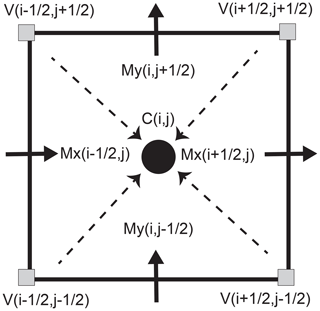Figure 1Setup of the staggered grid in 2-D. Variable C is located at the cell centre, V depicts variables located at cell vertices, and Mx and My represent variables located at cell mid-faces in the x or y direction.

We define temperature on the cell centres within our staggered grid. We discretise the temperature equation's advection term using a first-order upwind scheme, doing the physical time integration using either an implicit backward Euler or a Crank–Nicolson scheme. To ensure that our numerical results are not confounded by numerical diffusion, the grid Peclet number must be smaller than the physical Peclet number. Limiting numerical diffusion is one motivation for using high numerical resolution in our computations.

We rely on a pseudo-transient (PT) continuation or relaxation method to solve the system of coupled non-linear partial differential equations (Eq. 10) in an iterative and matrix-free way . To this end, we reformulate the thermomechanical Eq. (10) in a residual form:

$\begin{array}{}\text{(13)}& \begin{array}{rl}& -\frac{\partial {v}_{i}}{\partial {x}_{i}}={f}_{\mathrm{p}}\phantom{\rule{0.25em}{0ex}},\\ & \frac{\partial {\mathit{\tau }}_{ij}}{\partial {x}_{j}}-\frac{\partial P}{\partial {x}_{i}}+{F}_{i}={f}_{{\mathrm{v}}_{i}}\phantom{\rule{0.25em}{0ex}},\\ & -\frac{\partial T}{\partial t}-{v}_{i}\frac{\partial T}{\partial {x}_{i}}+\frac{{\partial }^{\mathrm{2}}T}{\partial {{x}_{i}}^{\mathrm{2}}}+{\mathit{\tau }}_{ij}{\stackrel{\mathrm{˙}}{\mathit{ϵ}}}_{ij}={f}_{\mathrm{T}}\phantom{\rule{0.25em}{0ex}}.\end{array}\end{array}$

The right-hand-side terms $\left({f}_{\mathrm{p}},{f}_{{\mathrm{v}}_{i}},{f}_{\mathrm{T}}\right)$ are the non-linear continuity, momentum and temperature residuals, respectively, and quantify the magnitude of the imbalance of the corresponding equations.

We augment the steady-state equations with PT terms using the analogy of physical transient processes such as the bulk compressibility or the inertial terms within the momentum equations . This formulation enables us to integrate the equation forward in pseudo-time τ until we reach the steady state (i.e. the pseudo-time derivatives vanish). Relying on transient physics within the iterative process provides well-defined (maximal) iterative time step limiters. We reformulate Eq. (10):

$\begin{array}{}\text{(14)}& \begin{array}{rl}& -\frac{\partial {v}_{i}}{\partial {x}_{i}}=\frac{\partial P}{\partial {\mathit{\tau }}_{p}}\phantom{\rule{0.25em}{0ex}},\\ & \frac{\partial {\mathit{\tau }}_{ij}}{\partial {x}_{j}}-\frac{\partial P}{\partial {x}_{i}}+{F}_{i}=\frac{\partial {v}_{i}}{\partial {\mathit{\tau }}_{{v}_{i}}}\phantom{\rule{0.25em}{0ex}},\\ & -\frac{\partial T}{\partial t}-{v}_{i}\frac{\partial T}{\partial {x}_{i}}+\frac{{\partial }^{\mathrm{2}}T}{\partial {{x}_{i}}^{\mathrm{2}}}+{\mathit{\tau }}_{ij}{\stackrel{\mathrm{˙}}{\mathit{ϵ}}}_{ij}=\frac{\partial T}{\partial {\mathit{\tau }}_{T}}\phantom{\rule{0.25em}{0ex}},\end{array}\end{array}$

where we introduced the pseudo-time derivatives $\partial /\partial \mathit{\tau }$ for the continuity $\left(\partial P/\partial {\mathit{\tau }}_{p}\right)$, the momentum $\left(\partial {\mathrm{v}}_{i}/\partial {\mathit{\tau }}_{{v}_{i}}\right)$ and the temperature $\left(\partial T/\partial {\mathit{\tau }}_{T}\right)$ equation.

For every non-linear iteration k, we update the effective viscosity ${{\mathit{\eta }}_{\mathrm{eff}}}^{\left[k\right]}$ in the logarithmic space by taking a fraction θη of the actual physical viscosity η[k] using the current strain rate and temperature solution fields and a fraction (1−θη) of the effective viscosity calculated in the previous iteration ${{\mathit{\eta }}_{\mathrm{eff}}}^{\left[k-\mathrm{1}\right]}$:

$\begin{array}{}\text{(15)}& {{\mathit{\eta }}_{\mathrm{eff}}}^{\left[k\right]}=\mathrm{exp}\left[{\mathit{\theta }}_{\mathit{\eta }}\mathrm{ln}\left({\mathit{\eta }}^{\left[k\right]}\right)+\left(\mathrm{1}-{\mathit{\theta }}_{\mathit{\eta }}\right)\mathrm{ln}\left({{\mathit{\eta }}_{\mathrm{eff}}}^{\left[k-\mathrm{1}\right]}\right)\right]\phantom{\rule{0.25em}{0ex}}.\end{array}$

We use the scalar ${\mathit{\theta }}_{\mathit{\eta }}\phantom{\rule{0.25em}{0ex}}\left(\mathrm{0}\le {\mathit{\theta }}_{\mathit{\eta }}\le \mathrm{1}\right)$ to select the fraction of a given non-linear quantity, here the effective viscosity ηeff, to be updated each iteration. When θη=0, we would always use the initial guess, while for θη=1, we would take 100% of the current non-linear quantity. We usually define theta to be in the range of ${\mathrm{10}}^{-\mathrm{2}}-{\mathrm{10}}^{-\mathrm{1}}$ in order to account for some time to fully relax the non-linear viscosity as the non-linear problem may not be sufficiently converged at the beginning of the iterations. This approach is in a way similar to an under-relaxation scheme and was successfully implemented in the ice-sheet model development by , for example.

The pseudo-time integration of Eq. (14) leads to the definition of pseudo-time steps $\mathrm{\Delta }{\mathit{\tau }}_{\mathrm{p}},\mathrm{\Delta }{\mathit{\tau }}_{{\mathrm{v}}_{i}}$ and ΔτT for the continuity, momentum and temperature equations, respectively. Transient physical processes such as compressibility (continuity equation) or acceleration (momentum equation) dictate the maximal allowed explicit pseudo-time step to be utilised in the transient process. Using the largest stable steps allows one to minimise the iteration count required to reach the steady state:

$\begin{array}{}\text{(16)}& \begin{array}{rl}\mathrm{\Delta }{\mathit{\tau }}_{\mathrm{p}}& =\frac{\mathrm{2.1}\phantom{\rule{0.25em}{0ex}}{n}_{\mathrm{dim}}{\mathit{\eta }}_{\mathrm{eff}}^{k}\left(\mathrm{1}+{\mathit{\eta }}_{\mathrm{b}}\right)}{max\left({n}_{\mathrm{i}}\right)}\phantom{\rule{0.25em}{0ex}},\\ \mathrm{\Delta }{\mathit{\tau }}_{{\mathrm{v}}_{i}}& =\frac{min\left(\mathrm{\Delta }{x}_{i}{\right)}^{\mathrm{2}}}{\mathrm{2.1}\phantom{\rule{0.25em}{0ex}}{n}_{\mathrm{dim}}{\mathit{\eta }}_{\mathrm{eff}}^{k}\left(\mathrm{1}+{\mathit{\eta }}_{\mathrm{b}}\right)}\phantom{\rule{0.25em}{0ex}},\\ \mathrm{\Delta }{\mathit{\tau }}_{\mathrm{T}}& ={\left(\frac{\mathrm{2.1}{n}_{\mathrm{dim}}}{min\left(\mathrm{\Delta }{x}_{i}{\right)}^{\mathrm{2}}}+\frac{\mathrm{1}}{\mathrm{\Delta }t}\right)}^{-\mathrm{1}}\phantom{\rule{0.25em}{0ex}},\end{array}\end{array}$

where ndim is the number of dimensions, and Δxi and ni are the grid spacing and the number of grid points in the i direction (i=x in 1-D, x,z in 2-D and $x,y,z$ in 3-D), respectively. The physical time step, Δt, advances the temperature in time. The pseudo-time step ΔτT is an explicit Courant–Friedrich–Lewy (CFL) time step that combines temperature advection and diffusion. Similarly, $\mathrm{\Delta }{\mathit{\tau }}_{{\mathrm{v}}_{i}}$ is the explicit CFL time step for viscous flow, representing the diffusion of strain rates with viscosity as the diffusion coefficient. It is modified to account for the numerical equivalent of a bulk viscosity ηb. We choose Δτp to be the inverse of $\mathrm{\Delta }{\mathit{\tau }}_{{\mathrm{v}}_{i}}$ to ensure that the pressure update is proportional to the effective viscosity, while the velocity update is sensitive to the inverse of the viscosity. This interdependence reduces the iterative method's sensitivity to the variations in the ice's viscosity.

During the iterative procedure, we allow for finite compressibility in the ice, $\partial P/\partial {\mathit{\tau }}_{\mathrm{p}}$, while ensuring that the PT iterations eventually reach the incompressible solution. The relaxation of the incompressibility constraint is analogous to the penalisation of pressure pioneered by and subsequently extended by others. Compared to projection-type methods, it has the advantage that no pressure boundary condition is necessary that will lead to numerical boundary layers . We use the parameter ηb to balance the divergence-free formulation of strain rates in the normal stress component evaluation, wherein it is multiplied with the pressure residual fp. Thus, normal stress is given by ${\mathit{\tau }}_{ii}=\mathrm{2}\mathit{\eta }\left({\stackrel{\mathrm{˙}}{\mathit{ϵ}}}_{ii}+{\mathit{\eta }}_{\mathrm{b}}{f}_{\mathrm{p}}\right)$. With convergence of the method, the pressure residual fp vanishes and the incompressible form of the normal stresses is recovered.

Combining the residual notation introduced in Eq. (13) with the pseudo-time derivatives in Eq. (14) leads to the update rules:

$\begin{array}{}\text{(17)}& \begin{array}{rl}{P}^{\left[k\right]}& ={P}^{\left[k-\mathrm{1}\right]}+\mathrm{\Delta }{P}^{\left[k\right]}\phantom{\rule{0.25em}{0ex}},\\ {{v}_{i}}^{\left[k\right]}& ={{v}_{i}}^{\left[k-\mathrm{1}\right]}+\mathrm{\Delta }{{v}_{i}}^{\left[k\right]}\phantom{\rule{0.25em}{0ex}},\\ {T}^{\left[k\right]}& ={T}^{\left[k-\mathrm{1}\right]}+\mathrm{\Delta }{T}^{\left[k\right]}\phantom{\rule{0.25em}{0ex}},\end{array}\end{array}$

where the pressure, velocity and temperature iterative increments represent the current residual [k] multiplied by the pseudo-time step:

$\begin{array}{}\text{(18)}& \begin{array}{rl}\mathrm{\Delta }{P}^{\left[k\right]}& =\mathrm{\Delta }{\mathit{\tau }}_{\mathrm{p}}{{f}_{\mathrm{p}}}^{\left[k\right]}\phantom{\rule{0.25em}{0ex}},\\ \mathrm{\Delta }{{v}_{i}}^{\left[k\right]}& =\mathrm{\Delta }{\mathit{\tau }}_{{\mathrm{v}}_{i}}{{f}_{{\mathrm{v}}_{i}}}^{\left[k\right]}\phantom{\rule{0.25em}{0ex}},\\ \mathrm{\Delta }{T}^{\left[k\right]}& =\mathrm{\Delta }{\mathit{\tau }}_{\mathrm{T}}{{f}_{\mathrm{T}}}^{\left[k\right]}\phantom{\rule{0.25em}{0ex}}.\end{array}\end{array}$

The straightforward update rule (Eq. 17) is based on a first-order scheme $\left(\partial /\partial \mathit{\tau }\right)$. In 1-D, it implies that one needs N2 iterations to converge to the stationary solution, where N stands for the total number of grid points. This behaviour arises because the time step limiter $\mathrm{\Delta }{\mathit{\tau }}_{{\mathrm{v}}_{\mathrm{i}}}$ implies a second-order dependence on the spatial derivatives for the strain rates. In contrast, a second-order scheme (Frankel1950), $\left(\mathit{\psi }{\partial }^{\mathrm{2}}/\partial {\mathit{\tau }}^{\mathrm{2}}+\partial /\partial \mathit{\tau }\right)$ invokes a wave-like transient physical process for the iterations. The main advantage is the scaling of the limiter as Δx instead of Δx2 in the explicit pseudo-transient time step definition. We can reformulate the velocity update as

$\begin{array}{}\text{(19)}& \begin{array}{rl}& \mathrm{\Delta }{{v}_{i}}^{\left[k\right]}=\mathrm{\Delta }{\mathit{\tau }}_{{\mathrm{v}}_{i}}{{f}_{{\mathrm{v}}_{i}}}^{\left[k\right]}\\ & +\left(\mathrm{1}-\frac{\mathit{\nu }}{{n}_{\mathrm{i}}}\right)\mathrm{\Delta }{{\mathrm{v}}_{i}}^{\left[k-\mathrm{1}\right]},\end{array}\end{array}$

where ψ can be expanded to $\left(\mathrm{1}-\mathit{\nu }/{n}_{i}\right)$ and acts like a damping term on the momentum residual. A similar damping approach is used for elastic rheology in the FLAC geotechnical software in order to significantly reduce the number of iterations needed for the algorithm to converge. The optimal value of the introduced parameter ν is found to be in a range $\left(\mathrm{1}\le \mathit{\nu }\le \mathrm{10}\right)$, and it is usually problem-dependent. This approach was successfully implemented in recent PT developments by and . The iteration count increases with the numerical problem size for second-order PT solvers and scales close-to-ideal multi-grid implementations. However, the main advantage of the PT approach is its conciseness and the fact that only one additional read/write operation needs to be included – keeping additional memory transfers to the strict minimum.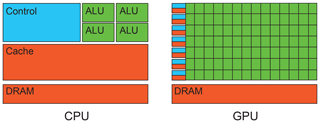Figure 2Schematic chip representation for both the central processing unit (CPU) and graphical processing unit (GPU) architecture. The GPU architecture consists of thousands of arithmetic and logical units (ALUs). On the CPU, most of the on-chip space is devoted to controlling units and cache memory, while the number of ALUs is significantly reduced.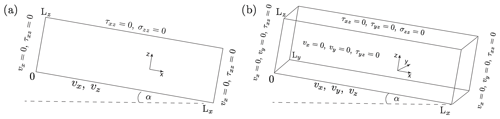Figure 3Model configuration for the numerical experiments: (a) 2-D model and (b) 3-D model. Both the surface and bed topography are flat but inclined at a constant angle of α. We show both the model coordinate axes and the prescribed boundary conditions.

Notably, the PT solution procedure leads to a two-way numerical coupling between temperature and deformation (mechanics), which enables us to recover an implicit solution to the entire system of non-linear partial differential equations. Besides the coupling terms, rheology is also treated implicitly; i.e. the shear viscosity η is always evaluated using the current physical temperature, T, and strain rate, ${\stackrel{\mathrm{˙}}{\mathit{ϵ}}}_{\mathrm{II}}$. Our method is fully local. At no point during the iterative procedure does one need to perform a global reduction, nor to access values that are not directly collocated. These considerations are crucial when designing a solution strategy that targets parallel hardware such as many-core GPU accelerators. We implemented the PT method in the MATLAB and CUDA C programming languages. Computations in CUDA C can be performed in both double- and single-precision arithmetic. The computations in CUDA C shown in the remainder of the paper were performed using double-precision arithmetic if not specified otherwise.

3 Leveraging hardware accelerators

## 3.1 Implementation on graphical processing units

Our GPU algorithm development effort is motivated by the aim to resolve the coupled thermomechanical system of equations (Eqs. 1213) with high spatial and temporal accuracy in 3-D. To this end, we exploit the low-level intrinsic parallelism of shared memory devices, particularly targeting GPUs. A GPU is a massively parallel device originally devoted to rendering the colour values for pixels on a screen independently from one another whereby the latency can be masked by high throughput (i.e. compute as many jobs as possible in a reasonable time). A schematic representation (Fig. 2) highlights the conceptual discrepancy between a GPU and CPU. On the GPU chip, most of the area is devoted to the arithmetic units, while on the CPU, a large area of the chip hosts scheduling and control microsystems.

The development of GPU-based solvers requires time to be devoted to the design of new algorithms that leverage the massively parallel potential of the current GPU architectures. Considerations such as limiting the memory transfers to the mandatory minimum, avoiding complex data layouts, preferring matrix-free solvers with low memory footprint and optimal parallel scalability instead of classical direct–iterative solver types are key in order to achieve optimal performance.

Our implementation does not rely on the CUDA unified virtual memory (UVM) features. UVM avoids the need to explicitly define data transfers between the host (CPU) and device (GPU) arrays but results in about 1 order of magnitude lower performance. We suspect the internal memory handling to be responsible for continuously synchronising the host and device memory, which is not needed in our case.

## 3.2 Multi-GPU implementation

We rely on a distributed memory parallelisation using the message passing interface (MPI) library to overcome the on-device memory limitation inherent to modern GPUs and exploit supercomputers' computing power. Access to a large number of parallel processes enables us to tackle larger computational domains or to refine grid resolution. We rely on domain decomposition to split our global computational domain into local domains, each executing on a single GPU handled by an MPI process. Each local process has its boundary conditions defined by (a) physics if on the global boundary or (b) exchanged information from the neighbouring process in the case of internal boundaries. We use CUDA-aware non-blocking MPI messages to exchange the internal boundaries among neighbouring processes. CUDA awareness allows us to bypass explicit buffer copies on the host memory by directly exchanging GPU pointers, resulting in an enhanced workflow pipelining. Our algorithm implementation and solver require no global reduction. Thus, there is no need for global MPI communication, eliminating an important potential scaling bottleneck. Although the proposed iterative and matrix-free solver features a high locality and should scale by construction, the growing number of MPI processes may deprecate the parallel runtime performance by about 20 % owing to the increasing number of messages and overall machine occupancy . We address this limitation by overlapping MPI communication and the computation of the inner points of the local domains using streams, a native CUDA feature. CUDA streams allow one to assign asynchronous kernel execution and thus enable the overlap between communication and computation, resulting in optimal parallel efficiency.

4 The model configuration

To verify the numerical implementation of the developed FastICE solver, we consider three numerical experiments based on a box inclined at a mean slope angle of α. We perform these numerical experiments on both 2-D and 3-D computational domains (Fig. 3a and b, respectively). The non-dimensional computational domains are ${\mathrm{\Omega }}_{\mathrm{2}-\mathrm{D}}=\left[\mathrm{0}{L}_{x}\right]×\left[\mathrm{0}{L}_{z}\right]$ and ${\mathrm{\Omega }}_{\mathrm{3}-\mathrm{D}}=\left[\mathrm{0}{L}_{x}\right]×\left[\mathrm{0}{L}_{y}\right]×\left[\mathrm{0}{L}_{z}\right]$ for 2-D and 3-D domains, respectively. The difference between the 2-D and the 3-D configurations lies in the boundary conditions imposed at the base and at the lateral sides. At the surface, the zero stress σijnj=0 boundary condition is prescribed in all experiments. Experiment 2's model configuration corresponds to the ISMIP benchmark , wherein experiment C relates to the 3-D case and experiment D relates to the 2-D case.

Table 1Experiments 1 and 2: non-dimensional model parameters and the dimensional values (D) for comparison.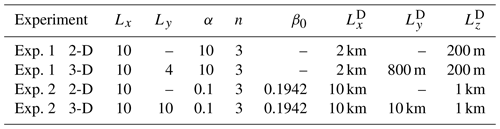Table 2Experiment 3: non-dimensional model parameters and the dimensional values (D) for comparison.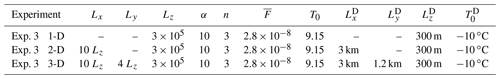Experiments 1 and 2 seek to first verify the implementation of the mechanical part of the Stokes solver, which is the computationally most expensive part (Eq. 8). For these experiments, we assume that the ice is isothermal and neglect temperature. We compare our numerical solutions to the solutions obtained by the commonly used finite-element Stokes solver Elmer/Ice , which has been thoroughly tested . Experiment 3 is a thermomechanically coupled case. The model parameters are the stress exponent n, the mean bed slope α, and the two horizontal distances Lx and Ly in their respective dimensions (x,y), which appear in Table 1. If a linear basal sliding law (Eq. 6) is prescribed, the respective 2-D and 3-D sliding coefficients are

$\begin{array}{}\text{(20)}& \begin{array}{rl}& {\mathit{\beta }}^{\mathrm{2}}\left(x\right)={\mathit{\beta }}_{\mathrm{0}}\left[\mathrm{1}+\mathrm{sin}\left(\frac{\mathrm{2}\mathit{\pi }x}{{L}_{x}}\right)\right]\phantom{\rule{0.25em}{0ex}},\\ & {\mathit{\beta }}^{\mathrm{2}}\left(x,y\right)={\mathit{\beta }}_{\mathrm{0}}\left[\mathrm{1}+\mathrm{sin}\left(\frac{\mathrm{2}\mathit{\pi }x}{{L}_{x}}\right)\mathrm{sin}\left(\frac{\mathrm{2}\mathit{\pi }y}{{L}_{y}}\right)\right]\phantom{\rule{0.25em}{0ex}},\end{array}\end{array}$

where β0 is a chosen non-dimensional constant. Differences may arise depending on the prescribed values for the parameters α, Lx, Ly and β0. Experiment 2 represents the ISMIP experiments C and D for L=10 km , but in our case using non-dimensional variables.

The mechanical part of Experiment 3 is analogous to Experiment 2. The boundary conditions are periodic in the x and y directions unless specified otherwise. The thermal problem requires additional boundary conditions in terms of temperature or fluxes. We set the surface temperature T0 to 0. At the bottom, we set the vertical flux qz to 0 and, on the sides, we impose periodic boundary conditions. The model parameters used in Experiment 3 are compiled in Table 2. We employ the semi-analytical 1-D model (Sect. 2.3) as an independent benchmark for the Experiment 3 calculations.

5 Results and performance

## 5.1 Experiment 1: Stokes flow without basal sliding

We compare our numerical solutions obtained with the GPU-based PT method using a CUDA C implementation (FastICE) to the reference Elmer/Ice model. We report all the values in their non-dimensional form, and the horizontal axes are scaled with their aspect ratio. We impose a no-slip boundary condition on all velocity components at the base and prescribe free-slip boundary conditions on all lateral domain sides. We prescribe a stress-free upper boundary in the vertical direction.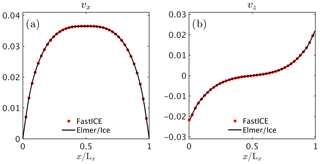Figure 4Comparison of the non-dimensional simulation results for the 2-D configuration of Experiment 1. We show (a) the horizontal component of the surface velocity, vx, and (b) the vertical component of surface velocity, vz, across the ice slab for both our FastICE model and Elmer/Ice. For context, the maximum horizontal velocity (vx≈0.0365) corresponds to ≈174 m yr−1. The horizontal distance is 2 km, while the ice thickness is 200 m. The box is inclined at 10.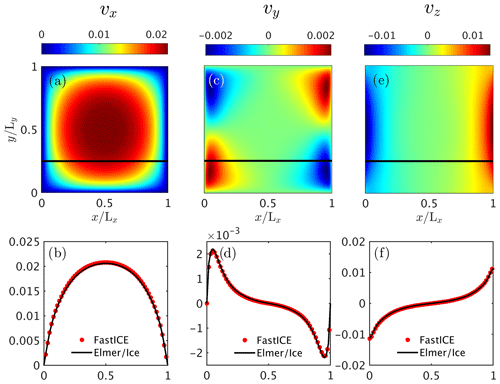Figure 5Non-dimensional simulation results for the 3-D configuration of Experiment 1. We report (a) the horizontal surface velocity component vx, (c) the horizontal surface velocity component vy and (e) the vertical surface velocity component vz. The black solid line depicts the position at which $y={L}_{y}/\mathrm{4}$. Panels (b), (d) and (f) show the surface velocity components vx,vy and vz, respectively, at $y={L}_{y}/\mathrm{4}$ and compare them against the results from the Elmer/Ice model.

In the 2-D configuration (Fig. 4), the horizontal velocity component vanishes at the left and right boundary, vx=0, and thus the maximum velocity values in the horizontal direction are located in the middle of the slab. On the left side ($x/{L}_{x}=\mathrm{0}$), the ice is pushed down (compression); thus, the vertical velocity values were negative. On the right side ($x/{L}_{x}=\mathrm{1}$), the ice is pulled up (extension), and the vertical velocity values were positive. Our FastICE results agree well with the numerical solutions produced by Elmer/Ice. The numerical resolution of the Elmer/Ice model is 1001×275 grid points in the x and z directions ($\approx \mathrm{8.25}×{\mathrm{10}}^{\mathrm{5}}$ degrees of freedom – DOFs), while we employed 2047×511 grid points ($\approx \mathrm{3.13}×{\mathrm{10}}^{\mathrm{6}}$ DOFs) within our PT method. We use higher numerical grid resolution within FastICE to jointly verify agreement with Elmer/Ice and convergence. The fact that we obtain matching results when increasing grid resolution significantly suggests that we have sufficiently resolved the relevant physical processes, even at relatively low resolution. We report an exception to this trend in the 3-D case of Experiment 2. The PT method's efficiency enables simulations with a large number of grid points without affecting the runtime. The DOFs represent three variables in 2-D (${v}_{x},{v}_{z},P$) and four variables in 3-D (${v}_{x},{v}_{y},{v}_{z},P$) multiplied by the number of grid points involved.

We find good agreement between the two model solutions in the 3-D configuration as well (Fig. 5). We employed a numerical grid resolution of $\mathrm{319}×\mathrm{159}×\mathrm{119}$ grid points in the x, y and z directions ($\approx \mathrm{2.41}×{\mathrm{10}}^{\mathrm{7}}$ DOFs) and used a numerical grid resolution of $\mathrm{61}×\mathrm{61}×\mathrm{21}$ ($\approx \mathrm{3.1}×{\mathrm{10}}^{\mathrm{5}}$ DOFs) in Elmer/Ice. Scaling our result to dimensional values (Table 1) results in a maximal horizontal velocity (vx) of ≈105 m yr−1. The horizontal distance is 2 km in the x direction and 800 m in the y direction, and the ice thickness is 200 m. The box is inclined at 10.

## 5.2 Experiment 2: Stokes flow with basal sliding

We then consider the case in which ice is sliding at the base (ISMIP experiments C and D). We prescribe periodic boundary conditions at the lateral boundaries and apply a linear sliding law at the base. The top boundary remains stress-free in the vertical direction.

We performed the 2-D simulation of Experiment 2 (Fig. 6) using a numerical grid resolution of 511×127 grid points ($\approx \mathrm{1.95}×{\mathrm{10}}^{\mathrm{5}}$ DOFs) for the FastICE solver and computed the Elmer/Ice solution using a numerical grid resolution of 241×120 ($\approx \mathrm{8.7}×{\mathrm{10}}^{\mathrm{4}}$ DOFs). We show both vx and vz velocity components at the slab's surface. The two models' results agree well.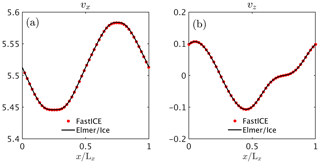Figure 6Non-dimensional simulation results for the 2-D configuration of Experiment 2. We plot (a) the horizontal surface velocity component vx and (b) the vertical surface velocity component vz across the slab for both our FastICE model and Elmer/Ice. In dimensional terms, the maximum horizontal velocity (vx≈5.58) corresponds to ≈16.9 m yr−1. The horizontal distance is 10 km, while the ice thickness is 1 km. The box is inclined at 0.1.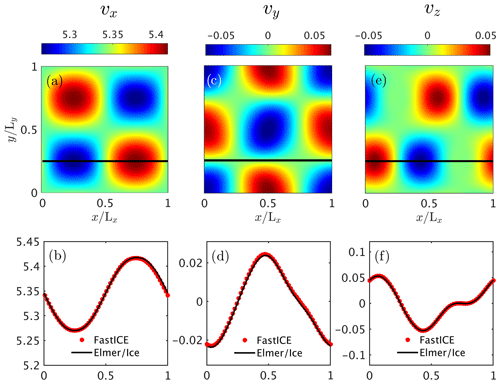Figure 7Non-dimensional simulation results for the 3-D configuration of Experiment 2. We report (a) the horizontal surface velocity component vx, (c) the horizontal surface velocity component vy and (e) the vertical surface velocity component vz. The black solid line depicts the position at which $y={L}_{y}/\mathrm{4}$. Panels (b), (d) and (f) show the surface velocity components vx,vy and vz, respectively, at $y={L}_{y}/\mathrm{4}$ and compare them against the results from the Elmer/Ice model.

We performed the 3-D simulation of Experiment 2 (Fig. 7) using a numerical grid resolution of $\mathrm{63}×\mathrm{63}×\mathrm{21}$ ($\approx \mathrm{3.33}×{\mathrm{10}}^{\mathrm{5}}$ DOFs) for our FastICE solver and a numerical grid resolution of $\mathrm{61}×\mathrm{61}×\mathrm{21}$ ($\approx \mathrm{3.12}×{\mathrm{10}}^{\mathrm{5}}$ DOFs) in the Elmer/Ice model. In dimensional units, the maximum horizontal velocity (vx) corresponds to ≈16.4 m yr−1. The horizontal distance is 10 km in the x direction 10 km in the y direction, and the ice thickness is 1 km. The box is inclined at 0.1.

We find good agreement between the two numerical implementations. Since the flow is mainly oriented in the x direction, the vy velocity component is more than 2 orders of magnitude smaller than the vx velocity component. Numerical errors in vy are more apparent than in the leading velocity component vx. We report a 1 order of magnitude increase in the time to solution in Experiment 2 compared to the Experiment 1 configuration owing to the periodicity on the lateral boundaries.

We employ a matching numerical resolution between FastICE and Elmer/Ice in this particular benchmark case. Using higher resolution for FastICE results in minor discrepancy between the two solutions, suggesting that the resolution in Fig. 7 is insufficient to capture small-scale physical processes. We discuss this issue more in Sect. 5.5 where we test the convergence of the FastICE numerical implementation upon grid refinement.Figure 8Non-dimensional simulation results for (a) the temperature deviation T and (b) the horizontal velocity component vx for the 1-D, 2-D and 3-D FastICE models at three different non-dimensional times 0.7×108, 1.4×108 and 1.9×108 compared to the 1-D reference model results. We employ a vertical grid resolution nz of 31,95 and 201 grid points. We sample the 1-D profiles at location $x={L}_{x}/\mathrm{2}$ in 2-D and at $x={L}_{x}/\mathrm{2}$ and $y={L}_{y}/\mathrm{2}$ in 3-D. The shaded areas correspond to the part of the solution that is above the melting temperature, since we do not account for phase transitions in this case.Figure 9Spatial distribution of (a) the temperature deviation from the initial temperature T and (b) the horizontal velocity component vx for 3-D (a) and 2-D (b) in non-dimensional units. We scale the domain extent with Lz. We compare the numerical solutions at non-dimensional times 0.7×108, 1.4×108 and 1.9×108.

## 5.3 Experiment 3a: thermomechanically coupled Stokes flow without basal sliding

We first verify that the 1-D, 2-D and 3-D model configurations from Experiment 3 produce identical results, assuming periodic boundary conditions on all lateral sides. In this case, all the variations in the x or y directions vanish ($\partial /\partial x$ and $\partial /\partial y$); thus, both the 2-D and 3-D models reduce to the 1-D problem. We employ a numerical grid resolution of $\mathrm{127}×\mathrm{127}×\mathrm{127}$ grid points in the x, y and z direction, 127×127 grid points in the x and z directions, and 127 grid points in the z direction for the 3-D, 2-D and 1-D problems, respectively.

We ensure that all results collapse onto the semi-analytical 1-D model solution (Sect. 2.3), which we obtained by analytically integrating the velocity field and solving the decoupled thermal problem separately (Eq. 11). From a computational perspective, we numerically solve Eq. (11) using a high spatial and temporal accuracy and therefore minimise the occurrence of numerical errors. We establish the 1-D reference solution for both the temperature and the velocity profile, solving Eq. (11) on a regular grid, reducing the physical time steps until we converge to a stable reference solution. Our reference simulation involves 4000 grid points and a non-dimensional time step of 5×105 (using a backward Euler time integration). We reach the total simulation time of 2.9×108 within 580 physical time steps.

We report overall good agreement of all model solutions (1-D, 2-D, 3-D and 1-D reference) at the three reported stages for this scenario (Fig. 8). As expected from the 1-D model solution, temperature varies only as a function of time and depth, with the highest value obtained close to the base and for longer simulation times. Similarly, the velocity profile is equivalent to the 1-D profile, and the largest velocity value is located at the surface. We only report the horizontal velocity component vx for the 2-D and the 3-D models, since vy and vz feature negligible magnitudes. Thus, we only observe spatial variation in the vertical z direction. We report the non-dimensional temperature T (Fig. 9a) and horizontal velocity vx (Fig. 9b) fields for both the 3-D and the 2-D configurations compared at non-dimensional time 0.7×108, 1.4×108 and 1.9×108. The dimensional results from Experiment 3 correspond to a 300 m thick ice slab inclined at a 10 angle with an initial surface temperature of −10C. The maximum initial velocity for the isothermal ice slab corresponds to ≈486 m yr−1, while the maximum velocity just before the melting point is reached corresponds to 830 m yr−1. The comparison snapshot times are 1.6, 3.2 and 4.4 years.

The semi-analytical 1-D solution enables us to evaluate the influence of the numerical coupling method and time integration and to quantify when and why high spatial resolution is required in thermomechanical ice-flow simulations. We compare the 1-D semi-analytical reference solution (Eq. 11) to the results obtained with the 1-D FastICE solver for three spatial numerical resolutions (nz=31, 95 and 201 grid points) at three non-dimensional times 1×108, 2×108 and 2.9×108 (Fig. 10). The grey area in Fig. 10 highlights where the melting temperature is exceeded. Since our semi-analytical reference solution does not include phase transitions, we also neglect this component in the numerical results. During the early stages of the simulation, the thermomechanical coupling is still minor, and solutions at all resolution levels are in good agreement with one another and with the reference. The low-resolution solution starts to deviate from the reference (Fig. 10b) when the coupling becomes more pronounced close to the thermal runaway point . The high-spatial-resolution solution is satisfactory at all stages. We conclude that high spatial resolution is required to accurately capture the non-linear coupled behaviour in regimes close to the thermal runaway, which is seldom the case in the models reported in the literature.

Thermomechanical strain localisation may significantly impact the long-term evolution of a coupled system. A recent study by suggested that partial coupling may result in underestimating the thermomechanical localisation compared to the fully coupled approach, as reported in their Fig. 8. We compare three coupling methods (Fig. 11): (1) a fully coupled implicit PT method, as described in the numerical section, whereby the viscosity and the shear heating term are implicitly determined by using the current guess; (2) an implicit numerically uncoupled mechanical and thermal model; and (3) an explicit numerically uncoupled mechanical and thermal model. The numerical time integration in physical time is performed using an implicit backward Euler method for (1) and (2) and a forward Euler explicit time integration method for (3). We utilise the identical non-dimensional time step for both the explicit and the implicit numerical time integration. We perform 580 time steps, reaching a simulation time of 2.9×108. We employ a vertical grid resolution of nz=201 grid points for all models. The chosen time step for the explicit integration of the heat diffusion equation is below the CFL stability condition given by Δz2∕2.1 in 1-D, where Δz represents the grid spacing in a vertical direction.

Physically, the viscosity and shear heating terms are coupled and are a function of temperature and strain rates, but we update the viscosity and the shear heating term based on temperature values from the previous physical time step. Thus, the shear heating term can be considered a constant source term in the temperature evolution equation during the time step, leading to a semi-explicit rheology. We show the 1-D numerical solutions of (blue) the fully coupled method with a backward Euler (implicit) time integration and the two uncoupled methods with either (green) backward (implicit) or (red) forward (explicit) Euler time integration (Fig. 11) and compare them to the 1-D reference model solution. Surprisingly, and in contrast to , we observe a good agreement between all methods, suggesting that the different coupling strategies capture the coupled flow physics with sufficient accuracy given high enough spatial and temporal resolution. However, for a longer-term evolution, the uncoupled approaches may predict lower temperature and velocity values than the fully coupled approach.Figure 10Non-dimensional simulation results for (a) the temperature deviation T and (b) the horizontal velocity component vx to test solver performance at three resolutions. The vertical resolutions are LR=31, MR=95 and HR=201 grid points for low-, mid- and high-resolution runs, respectively. We compare the results for non-dimensional time 1×108, 2×108 and 2.9×108. The shaded areas correspond to the part of the solution that is above the melting temperature, since we do not account for phase transitions in this benchmark.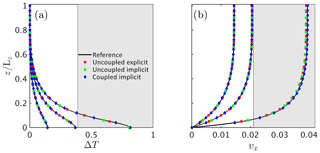Figure 11Non-dimensional simulation results for (a) the temperature deviation T and (b) the horizontal velocity component vx to evaluate different numerical time integration schemes. We consider three non-dimensional times 1×108, 2×108 and 2.9×108 and compare our numerical estimates to the reference model. As before, the shaded areas correspond to the part of the solution that is above the melting temperature, since we neglect phase transitions in this comparison.

## 5.4 Experiment 3b: thermomechanically coupled Stokes flow in a finite domain

Boundary conditions corresponding to immobile regions in the computational domain may induce localisation of deformation and flow observed in locations such as shear margins, grounding zones or bedrock interactions. Dimensionality plays a key role in such configurations, causing the stress distribution to be variable among the considered directions.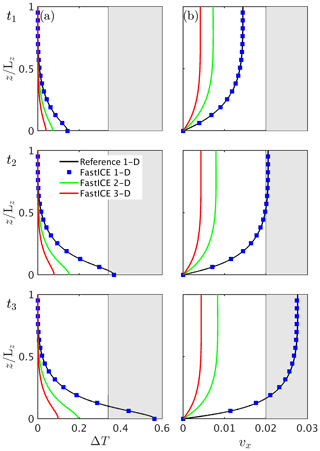Figure 12Non-dimensional simulation results for (a) the temperature deviation T and (b) the horizontal velocity component vx for the 1-D, 2-D and 3-D FastICE models at three non-dimensional times 1×108, 2×108 and 2.5×108 compared to our analytical solution. We sample the 1-D profiles at location $x={L}_{x}/\mathrm{2}$ in 2-D and at $x={L}_{x}/\mathrm{2}$ and $y={L}_{y}/\mathrm{2}$ in 3-D. The shaded area corresponds to the part of the solution that is above the melting temperature, approximately 0.35 of the temperature deviation.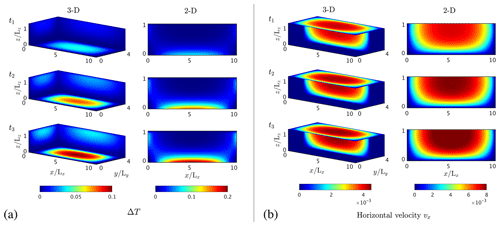Figure 13Non-dimensional simulation results of (a) the temperature deviation from the initial temperature T and (b) the horizontal velocity component vx for Experiment 3 at three non-dimensional times 1×108, 2×108 and 2.5×108 for both the 2-D and 3-D configurations.

We used the configuration in Experiment 3 to investigate the spatial variations in temperature and velocity distributions by defining no-slip conditions on the lateral boundaries for the mechanical problem and prescribing zero heat flux through those boundaries. We employ a numerical grid resolution of $\mathrm{511}×\mathrm{255}×\mathrm{127}$ grid points, 511×127 grid points and 201 grid points for the 3-D, 2-D and 1-D case, respectively. We prescribe a non-dimensional time step of 5×105. We perform 500 numerical time steps and reach a total non-dimensional simulation time of 2.5×108. We then compare the temperature T and horizontal velocity component vx at three times obtained with the 1-D, 2-D and 3-D FastICE solver to the reference solution (Fig. 12). We use 1-D profiles for comparison, taken at location $x={L}_{x}/\mathrm{2}$ in the 2-D model and at location $x={L}_{x}/\mathrm{2}$ and $y={L}_{y}/\mathrm{2}$ in the 3-D model. We also report the temperature variation ΔT (Fig. 13a) and the horizontal velocity component vx (Fig. 13b) for both the 2-D and 3-D simulations. The melting temperature approximately corresponds to 0.35 of the temperature deviation. The reported results correspond to a 2.3-, 4.6- and 5.8-year evolution.

All three models start with identical initial conditions for the thermal problem, i.e. ΔT=0 throughout the entire ice slab. The difference between the models arises owing to different stress distributions in 1-D, 2-D or 3-D. For instance, the additional stress components inherent in 2-D and 3-D are of the same order of magnitude as the 1-D shear stress for the considered aspect ratio, reducing the horizontal velocity vx in the 2-D and 3-D models. This also impacts the shear heating term, reducing the source term in the temperature evolution equation. In the 1-D configuration, the unique shear stress tensor component is a function only of depth. On the other endmember, the 3-D configurations allow for a spatially more distributed stress state. They lower strain rates in this scenario and reduce the magnitude of shear heating in higher dimensions. The spatially heterogeneous temperature and strain rate fields in all directions require the utilisation of sufficiently high spatial numerical resolution in all directions in order to accurately resolve spontaneous localisation.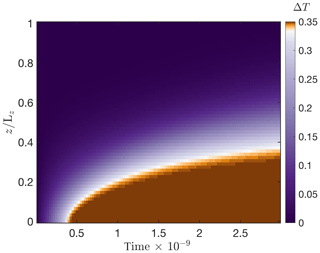Figure 14Experiment 3 includes a phase transition owing to melting. We report the evolution in time of non-dimensional temperature variation ΔT along a vertical profile picked at location $x={L}_{x}/\mathrm{2}$ within a 2-D run from Experiment 3. For this purpose, we run the 2-D FastICE models from Experiment 3 for a duration of 2.9×109.

We did not consider phase transition in the previous experiments for the sake of model comparison and because the analytical solution excluded this process. The existence of a phase transition caps the temperature at the pressure melting point in regions with pronounced shear heating, as illustrated in 2-D in Fig. 14. The simulation represents the thermomechanically coupled Experiment 3 with no sliding and thermally impermeable walls (similar to Fig. 13). Meltwater production consumes excess heat generated by shear heating. Thus, melting provides a physical mechanism that avoids thermal runaway in shear-heating-dominated zones in the ice. The experiment duration in dimensional units is 70 years, and the maximal temperature increase is 10 C upon reaching the melting point.

## 5.5 Verification of the FastICE numerical implementation

In order to confirm the accuracy of the FastICE numerical implementation, we report truncation errors (L2 norms) upon numerical grid refinement. We consider both the 2-D and 3-D configurations of Experiment 2 for this convergence test. We vary the numerical grid resolution, keeping the relative grid step Δx and Δy (and Δz in 3-D) ratio. We utilise a high-resolution numerical simulation as a reference and perform three additional simulations in which we keep dividing the number of grid points in both the x and y (and z in 3-D) direction by a factor of 2. We report the L2 norms,

$\begin{array}{}\text{(21)}& \begin{array}{rl}& ||{P}_{\mathrm{err}}|{|}_{\mathrm{2}}=||{P}_{\mathrm{ref}}-{P}_{\mathrm{coarse}}|{|}_{\mathrm{2}}\phantom{\rule{0.25em}{0ex}},\\ & ||{{v}_{x}}_{\mathrm{err}}|{|}_{\mathrm{2}}=||{{v}_{x}}_{\mathrm{ref}}-{{v}_{x}}_{\mathrm{coarse}}|{|}_{\mathrm{2}}\phantom{\rule{0.25em}{0ex}},\end{array}\end{array}$

for both the pressure P and the horizontal downslope vx velocity component on a logarithmic plot for both the 2-D (Fig. 15a) and 3-D configurations (Fig. 15b). The FastICE numerical implementation converges with increasing numerical resolution, and we report linear fitting slopes of −1.19 for pressure and about −1.4 for horizontal the velocity component.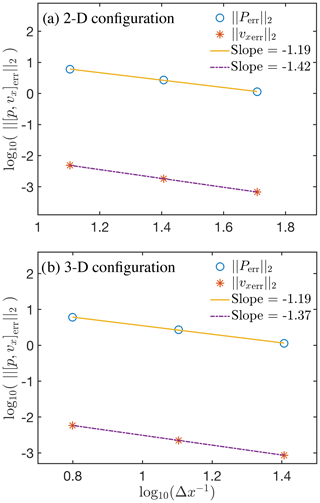Figure 15Evolution of velocity and pressure truncation errors (L2 norm) upon grid refinement for (a) the 2-D configuration and (b) the 3-D configuration of Experiment 2.

We additionally report the behaviour of the residuals' converge as a function of the non-linear iterations ${n}_{\mathrm{iter}}^{\mathrm{nonlin}}$ for the FastICE GPU-based implementation (Fig. 16a). The reported convergence history stands for a 2-D configuration of Experiment 3 and a numerical grid resolution of 511×127 grid points. The optimal damping parameter used in this case is ν=2 (Eq. 19). We further report the sensitivity of the accelerated PT scheme on the damping parameter ν (Fig. 16b). We show that selecting the optimal damping parameter (in the reported case ν=2) ensures a relatively low number of iterations to converge both the linear and non-linear thermomechanical problem.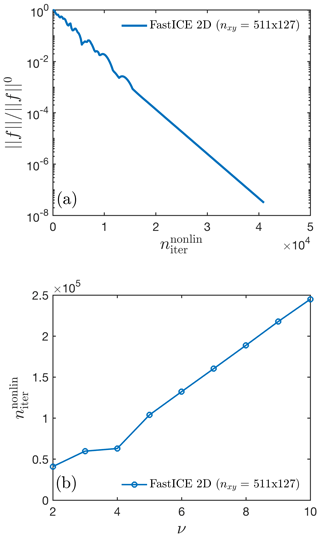Figure 16Residual evolution and convergence efficiency of the 2-D FastICE GPU-based implementation for a numerical grid resolution of 511×127 grid points targeting a relative non-linear tolerance of ${\mathrm{tol}}_{\mathrm{nonlin}}=\mathrm{1}e-\mathrm{8}$. (a) Relative total non-linear residuals $f=max\left({f}_{\mathrm{P}},{f}_{{\mathrm{v}}_{i}},{f}_{\mathrm{T}}\right)$ as a function of non-linear iterations and (b) the non-linear iteration count as a function of the damping parameter ν (Eq. 19).

## 5.6 The computational performance

We used two metrics to assess the performance of the developed FastICE PT algorithm: the effective memory throughput (MTPeff) and the wall time. We first compare the effective memory throughput of the vectorised MATLAB CPU implementation and the single-GPU CUDA C implementation. We employ double-precision (DP) floating-point arithmetic in CUDA C for fair comparison. Second, we employ the wall-time metric to compare the performance of our various implementations (MATLAB, CUDA C) and compare these to the time to solution of the Elmer/Ice solver.

We use two methods to solve the linear system in Elmer/Ice. In the 2-D experiments, we use a direct method, and in 3-D, we use an iterative method. The direct method used in 2-D relies on the UMFPACK routines to solve the linear system. To solve the 3-D experiments, we employ the available bi-conjugate gradient-stabilised method (BICGstab) with an ILU0 preconditioning. We employ the configuration in Experiment 1 for all the performance measurements. We use an Intel i7 4960HQ 2.6 GHz (Haswell) four-core CPU to benchmark all the CPU-based calculations. For simplicity, we only ran single-core CPU tests, staying away from any CPU parallelisation of the algorithms. Thus, our MATLAB or the Elmer/Ice single-core CPU results are not representative of the CPU hardware capabilities and are only reported for reference.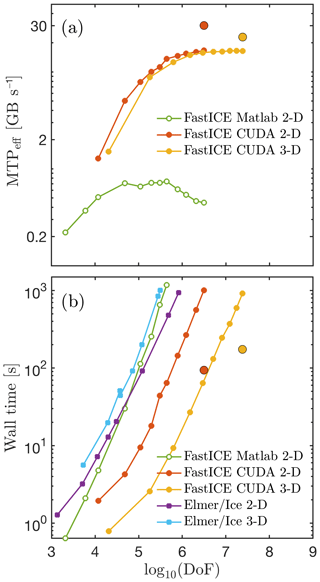Figure 17Performance evaluation of the FastICE mechanical solver in terms of (a) the effective memory throughput MTPeff in GB per second and (b) the wall time (in seconds) to converge the Stokes solver to a relative non-linear tolerance of ${\mathrm{tol}}_{\mathrm{nonlin}}={\mathrm{10}}^{-\mathrm{8}}$. We report the results obtained using a 2-D CPU-based single-core vectorised MATLAB implementation of FastICE, a 2-D and 3-D GPU-based CUDA C implementation of FastICE, and a 2-D (direct) and 3-D (iterative) solver within the Elmer/Ice FEM single-core CPU-based model. The CPU codes are executed on an Intel i7 4960HQ CPU processor with 8 GB of RAM, and the GPU codes are launched on a Nvidia Titan X (Maxwell) GPU with 12 GB of on-board memory. All the computations are performed in double-precision arithmetic, with the only exception for the two single-precision GPU-based runs depicted with larger red (2-D) and orange (3-D) symbols. The single-core FastICE CPU MATLAB and Elmer/Ice results are shown for reference; they are not meant for performance comparison because we did not enable multi-threading in MATLAB and did not have access to a parallel version of Elmer/Ice.

The FastICE PT solver relies on evaluating a finite-difference stencil. Each cell of the computational domain needs to access neighbouring values in order to approximate derivatives. These memory access operations are the performance bottleneck of the algorithm, making it memory-bounded. Thus, the algorithm's performance depends crucially on the memory transfer speed, and not the rate of the floating-point operations. Memory-bounded algorithms place additional pressure on modern many-core processors, since the current chip design tends toward large flop-to-byte ratios. Over the past years and decades, the memory bandwidth increase has been much slower compared to the increase in the rate of floating-point operations.

As shown by and , a relevant metric to assess the performance of memory-bounded algorithms is the effective memory throughput (MTPeff) (Eq. 22). The MTPeff determines how efficiently data are transferred between the main memory and the arithmetic units and is inversely proportional to the execution time:

$\begin{array}{}\text{(22)}& {\mathrm{MTP}}_{\mathrm{eff}}=\frac{\left({n}_{x}{n}_{y}{n}_{z}\right){n}_{\mathrm{iter}}\phantom{\rule{0.25em}{0ex}}{n}_{\mathrm{IO}}\phantom{\rule{0.25em}{0ex}}{n}_{\mathrm{p}}}{{\mathrm{1024}}^{\mathrm{3}}\phantom{\rule{0.25em}{0ex}}{t}_{\mathrm{nt}}}\phantom{\rule{2em}{0ex}}\left[\text{GB}\phantom{\rule{0.125em}{0ex}}{\text{s}}^{-\mathrm{1}}\right],\end{array}$

where (nxnynz) stands for the total number of grid points, niter is the total number of numerical iterations performed, np is the arithmetic precision (single – 4 bytes or double – 8 bytes), tnt is the wall time in seconds needed to compute the niter iterations and nIO is the performed number of memory accesses. It represents the minimum number of memory operations (read-and-write or read only) required to solve a given physical problem. For instance, in the mechanical Stokes solver for Experiment 1, we have to update (read-and-write) three arrays (vx,vz and P) at every iteration in 2-D and four arrays (${v}_{x},{v}_{y},{v}_{z}$ and P) at every iteration in 3-D. Thus, the update of the mandatory arrays requires a minimum of six (eight) read-and-write operations in 2-D (3-D). One additional read-and-write is needed to resolve the non-linear viscosity; thus, nIO=10 in the 2-D case and nIO=12 in 3-D.

We report MTPeff values obtained with the FastICE algorithm for both the vectorised MATLAB (CPU) and the CUDA C (GPU) implementations in double-precision arithmetic (Fig. 17a). We also show the GPU performance using single-precision arithmetic (Fig. 17a – green diamonds). The results we obtain should be compared to the peak memory throughput value MTPpeak for the specific hardware used. The MTPpeak reports the memory transfer rates delivered only by performing memory copy operations with no computations. This value reflects the hardware performance limit and the maximal effective memory bandwidth. We measure MTPpeak values for the Intel i7 4960HQ CPU of 20 GB s−1 and of 260 GB s−1 for the Nvidia Titan X GPU. The single-core vectorised MATLAB CPU implementation achieves about 0.7 GB s−1, and the CUDA C implementation 16 GB s−1. Thus, the MATLAB single-core CPU implementation reaches 3.5 % of the (CPU) hardware peak value, and the CUDA C (GPU) implementation at about 6.15 % and 11 % of the (GPU) hardware peak value using double-precision and single-precision arithmetic, respectively. Further improvement of the GPU MTPeff values can be achieved by optimising the GPU code using more on-the-fly calculations and advanced kernel scheduling.

We investigate the wall time to solve one time step with the FastICE GPU solver for both the 2-D and the 3-D configurations (Fig. 17b). We found wall times of about 15 min to solve $\approx \mathrm{2.4}×{\mathrm{10}}^{\mathrm{7}}$ DOFs with double-precision arithmetic and only 3 min when using single-precision arithmetic on a Nvidia Titan X (Maxwell) GPU. In future investigations, one may consider comparing wall times obtained by CPU algorithms fully enabling all cores of the CPU against wall times for GPUs within the same price and power consumption range.

The 3-D performance results obtained on various available Nvidia GPUs are summarised in Fig. 18. We performed all the calculations using double-precision arithmetic. We compare the MTPeff and wall-time values as functions of the DOFs. We tested GPUs from various price ranges and chip generations, targeting entry-level GPUs such as the Nvidia Quadro P1000 (Pascal), high-end gaming cards such as the Nvidia Titan Black (Kepler) or the Nvidia Titan X (Maxwell), and data-centre-class GPU accelerators such as the Nvidia Tesla V100 PCIe (Volta). The MATLAB implementation peak MTPeff values are about 0.46 GB s−1, the Quadro P1000 (Pascal) values about 4.3 GB s−1, the Titan Black (Kepler) 12.4 GB s−1, the Titan X (Maxwell) 16.7 GB s−1 and the Tesla V100 (Volta) 83.2 GB s−1. The MTPeff values directly impact the wall time, since the memory bandwidth is the bottleneck in FastICE. We solved a 3-D problem involving $\mathrm{511}×\mathrm{255}×\mathrm{127}$ grid points (6.6×107 DOFs) in about 1 h on the Titan Black GPU, 40 min on the Titan X GPU, and only 8 min on the Tesla V100 GPU. Notably, at this resolution, we employed about 4.5 GB of memory to solve the isothermal Stokes model. The results suggest that more recent GPUs such as the data-centre Tesla V100 (Volta) offer a more significant (order of magnitude higher) performance increase than entry-level GPU accelerators, such as the Quadro P1000.

We share the performance of the GPU-MPI implementation of FastICE to execute on distributed memory machines. We achieve a weak scaling parallel efficiency of 99 % on the 512 Nvidia K80 (Kepler) GPUs on the Xstream Cray CS-Storm GPU compute cluster at the Stanford Research Computing Facility. As our baseline, we use a non-MPI single-GPU calculation. We then repeat the experiment using 1 to 512 MPI processes (thus GPUs) and report the normalised execution time (Fig. 19). The effective drop in parallel efficiency is only 1 % involving 1 to 512 MPI processes. We achieve this close-to-optimal parallel efficiency by overlapping MPI message communication and local domain stencil calculations. We specifically employ distinct CUDA streams in order to execute the communication and computation overlap asynchronously. We repeat a similar experiment on both the Volta node, an 8 Nvidia Tesla V100 32 GB (Nvlink Volta) GPU compute node (analogous to Nvidia's DGX-1 box), and the octopus supercomputer hosting 128 consumer electronics Nvidia Titan X (Maxwell) GPUs at the Swiss Geocomputing Centre, University of Lausanne, Switzerland. On the Volta node, we report a weak scaling parallel efficiency of 0.985 % for a single MPI process running at 0.99 % of the non-MPI reference. On the octopus supercomputer, we report a parallel efficiency of 95.5 % with an effective drop in parallel efficiency of only 2 % involving 1 to 128 MPI processes.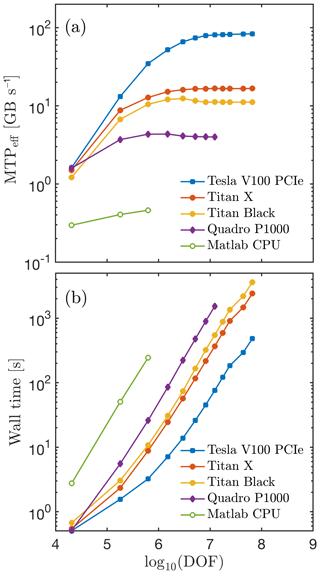Figure 18Performance evaluation of the FastICE mechanical solver in terms of (a) effective memory throughput MTPeff in GB per second and (b) wall time (in seconds) to converge the Stokes solver to a relative non-linear tolerance of ${\mathrm{tol}}_{\mathrm{nonlin}}={\mathrm{10}}^{-\mathrm{8}}$. We report the results from a 3-D CPU-based single-core vectorised MATLAB implementation and a 3-D GPU-based CUDA C implementation of FastICE running on different GPU chip architectures. The CPU codes are executed on an Intel i7 4960HQ CPU processor with 8 GB of RAM. The GPU codes were launched on a Nvidia Titan Black (Kepler) GPU with 6 GB, a Nvidia Titan X (Maxwell) GPU 12 GB, a Nvidia Quadro P1000 (Pascal) 4 GB and a Nvidia Tesla V100 PCIe (Volta) 32 GB.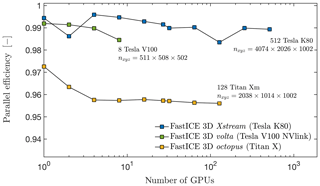Figure 19MPI weak scaling of the 3-D thermomechanically coupled GPU-based FastICE software. We report the parallel efficiency (–) of the numerical application on three different Nvidia hardware accelerators, the 1-512 Tesla K80 12 GB data-centre GPUs, the 1-8 Tesla V100 32 GB Nvlink data-centre GPUs and the 1-128 Titan X (Maxwell) 12 GB consumer electronics GPUs. These accelerators are available via the Xstream supercomputer, the Volta node and the octopus supercomputer, respectively. Note that the execution time baseline used to compute the parallel efficiency represents a non-MPI calculation. We report the highest numerical grid resolution nxyz achieved on each distributed memory machine.

6 Discussion

Numerically resolving thermomechanical processes in ice is vital for improving our understanding of the physical processes that govern the transition from fast to slow ice in a changing climate. To date, very few studies have investigated the numerical aspects of thermomechanically coupled Stokes solvers (e.g. Duretz et al.2019). Existing assessments (e.g. Zhang et al.2015) usually employ low spatial resolution and do not address the influence of the numerical implementation of multi-physics coupling strategies or the role of numerical time integration. To avoid the significant computational expense of a thermomechanically coupled full Stokes model, many studies relied either on the computationally less expensive shallow ice approximations, linear or linearised Stokes models, or low spatial resolutions. None of the approaches have resolved the multi-physics and multiscale processes governing the boundaries of streaming ice, including shear margins, grounding zones and the basal interface.

To address these limitations, we have developed FastICE, a new parallel GPU-based numerical model. The goal of FastICE is to better understand the physical processes that govern englacial instabilities such as thermomechanical localisation at the field site, rather than the regional, scale. It hence targets different scientific problems than many existing land–ice models and complements these previous models. FastICE is based on an iterative pseudo-transient finite-difference method. Our discretisation yields a concise matrix-free algorithm well suited to use the intrinsic parallelism of modern hardware accelerators such as GPUs. Our choices enable high-resolution 2-D and 3-D thermomechanically coupled simulations to efficiently perform on desktop computers and to scale linearly on supercomputers, both featuring GPU accelerators.

The significant temperature dependence of ice's shear viscosity leads to pronounced spatial variations in the viscosity, which affects the convergence rate of our iterative PT method. Resolving shear flow localisation is challenging in this context, since it requires the simultaneous minimisation of errors in locations of the computational domain that are governed by different characteristic timescales. Our PT approach allows us to capture the resulting spatial heterogeneity and offers a physically motivated strategy to locally ensure the stability of the iterative scheme using local pseudo-time steps, analogous to diagonal preconditioning in matrix-based direct approaches. The conciseness and simplicity of the implementation allows us to explore influences of various coupling methods and time integrations in a straightforward way. The PT approach is an interesting choice for educational purposes and research problems given its conciseness and efficiency, respectively.

We quantify the scalability of our approach through extensive performance tests, whereby we investigated both the time to solution and the efficiency of exploiting the current hardware capabilities at their maximal capacities. To verify the accuracy and the coherence of the proposed results, we performed a set of benchmark experiments, obtaining excellent agreement with results from the widely used glacier flow model Elmer/Ice. Experiment 3 verifies that, under the assumption of periodic configurations, the 1-D, 2-D and 3-D models return matching results.

Further, we have tested the accuracy of our numerical solutions for different time integration schemes, including forward (explicit) and backward (implicit) Euler and different physical time steps. The value of the numerical time step must be chosen as sufficiently small so as to resolve the relevant physical processes. We limited the maximal time step in the explicit time integration scheme by the CFL stability criterion for temperature diffusion. For high spatial numerical resolutions, the CFL-based time step restriction is sufficient to resolve the coupled thermomechanical process. However, this conclusion is not valid for low spatial resolutions (e.g. fewer than 20 grid points). At low resolution, the CFL-based stability condition predicts time step values larger than the non-dimensional time (2×108) needed to raise the temperature. Thus, we did not sufficiently resolve the physical process. An implicit scheme for the time integration remedies the stability issue but does not guarantee accuracy. Independent of the numerical time integration scheme used, the range of time step values that resolve the coupled physics is close to the explicit stability criterion.

Our multi-GPU implementation of the thermomechanical FastICE solver achieves a close-to-ideal parallel efficiency featuring a runtime drop of only 1 % and 2 % compared to a single MPI process execution on 1-512 Nvidia K80 GPUs and on 1-128 Nvidia Titan X (Maxwell) GPUs, respectively (representing a 1 % and 4.5 % deviation from a non-MPI single-GPU runtime). We achieve this optimal domain decomposition parallelisation by overlapping communication and computation using native CUDA streams. This CUDA feature enables asynchronous compute kernel execution. Similar implementation and parallel scaling results were recently reported for hydromechanical couplings . Discrepancies in the parallel efficiency among the three tested distributed memory machines mainly result from the various hardware type and age, as well as from the interconnect specifications. The Xstream supercomputer features Nvidia Tesla K80 GPUs based on Kepler chip architecture launched in late 2014 as well as single-rail Mellanox FDR Infiniband interconnect. The octopus supercomputer features consumer electronics Nvidia Titan X GPUs based on the Maxwell chip architecture launched in mid-2015 as well as dual-rail Mellanox FDR Infiniband interconnect. The Volta node features the latest Nvidia Tesla V100 GPUs based on Volta chip architecture launched in mid-2018 and Nvlink technology as intra-node interconnect. More recent chip architectures reduce the relative computation time and may provide less room for hiding the MPI communication. Dual-rail interconnect doubles the inter-node throughput and thus reduces the communication time among distinct compute nodes. Note that Xstream features 16 GPUs per node, which may reduce the inter-node communication compared to octopus that features 4 GPUs per node.

7 Conclusions

In this study, we develop FastICE, an iterative solver that efficiently exploits the capabilities of modern hardware accelerators such as GPUs. We achieve rapid execution times on single GPUs monitoring and optimising memory transfers. We achieve close-to-ideal parallel efficiency (99 % and 95.5 %) on a weak scaling test up to 512 and 128 GPUs on heterogenous hardware by overlapping MPI communication and computations. The technical advances and utilisation of GPU accelerators enable us to resolve thermomechanically coupled ice flow in 3-D at high spatial and temporal resolution.

We benchmark the mechanical solver of FastICE against the community model Elmer/Ice, focusing specifically on explicit as opposed to implicit coupling and time integration strategies. We find that the physical time step must be chosen with care. Sufficiently high temporal resolution is necessary in order to accurately resolve the coupled physics. Although minor differences arise among uncoupled and coupled approaches, we observe less localisation for uncoupled models compared to the fully coupled ones. In additional to high temporal resolution, a relatively high spatial numerical resolution of more than 100 grid points in the vertical direction is necessary to resolve thermomechanical localisation for typical ice-sheet thicknesses on the order of hundreds of metres. The presented models enable us to gain further process-based understanding of ice-flow localisation. Resolving the coupled processes at very high spatial and temporal resolutions provides future avenues to address current challenges in accurately predicting ice-sheet dynamics.

Code availability

The FastICE software developed in this study is licensed under the GPLv3 free software licence. The latest version of the code is available for download from Bitbucket at: https://bitbucket.org/lraess/fastice/ (last access: 2 March 2020) and from: http://wp.unil.ch/geocomputing/software/ (last access: 2 March 2020). Past and future FastICE versions are available from a permanent DOI repository (Zenodo) at: https://doi.org/10.5281/zenodo.3461171 . The FastICE software includes code examples based on the PT method in both the MATLAB and CUDA C programming languages. The GPU routines run on a CUDA-capable GPU device. The multi-GPU version of the 3-D code requires CUDA-aware MPI to be installed. On the octopus GPU supercomputer, we installed CUDA 10.0 and built Open MPI 2.1.5 with CUDA 10.0 and GCC 6.5 on a CentOS 6.9 system.

Author contributions

LR participated in the early model and numerical method development stages, implemented the MPI version of the code, performed the scaling analysis, and reshaped the final version of the paper. AL realised the first version of the study, performed the benchmarks, and drafted the paper outline as the second chapter of his PhD thesis. FH and YP supervised the early stages of the study. JS contributed to the capped thermal model and provided feedback on the paper in the final stage. All authors have reviewed and approved the final version of the paper.

Competing interests

The authors declare that they have no conflict of interest.

Acknowledgements

We thank Samuel Omlin, Thibault Duretz and Mathieu Gravey for their technical and scientific support. We thank Thomas Zwinger and two anonymous reviewers for valuable comments, which enhanced the study. We acknowledge the Swiss Geocomputing Centre for computing resources on the octopus supercomputer and are grateful to Philippe Logean for continuous technical support. Ludovic Räss acknowledges support from the Swiss National Science Foundation's Early Postdoc Mobility Fellowship 178075. This research was supported by the National Science Foundation through the Office of Polar Programs awards PLR-1744758 and PLR-1739027. This material is based upon work supported in part by the U.S. Army Research Office under contract/grant number W911NF-12-R0012-04 (PECASE). This work used the XStream computational resource, supported by the National Science Foundation Major Research Instrumentation programme (ACI-1429830).

Financial support

This research has been supported by the Swiss National Science Foundation's Early Postdoc Mobility Fellowship (grant no. 178075), the National Science Foundation through the Office of Polar Programs (grant nos. PLR-1744758 and PLR-1739027), and the The U.S. Army Research Laboratory and the U.S. Army Research Office (grant no. W911NF-12-R0012-04). This work used the XStream computational resource, supported by the National Science Foundation Major Research Instrumentation programme (ACI-1429830).

Review statement

This paper was edited by Alexander Robel and reviewed by two anonymous referees.

References

Bassis, J.: Hamilton-type principles applied to ice-sheet dynamics: new approximations for large-scale ice sheet flow, J. Glaciol., 97, 497–513, 2010. a

Brædstrup, C., Damsgaard, A., and Egholm, D. L.: Ice-sheet modelling accelerated by graphics cards, Comput. Geosci., 72, 210–220, 2014. a

Brinkerhoff, D. J. and Johnson, J. V.: Data assimilation and prognostic whole ice sheet modelling with the variationally derived, higher order, open source, and fully parallel ice sheet model VarGlaS, The Cryosphere, 7, 1161–1184, https://doi.org/10.5194/tc-7-1161-2013, 2013. a

Brinkerhoff, D. J. and Johnson, J. V.: Dynamics of thermally induced ice streams simulated with a higher-order flow model, J. Geophys. Res.-Earth, 120, 1743–1770, 2015. a

Bueler, E. and Brown, J.: Shallow shelf approximation as a “sliding law” in a thermomechanically coupled ice sheet model, J. Geophys. Res., 114, F03008, https://doi.org/10.1029/2008JF001179, 2009. a, b

Bueler, E., Brown, J., and Lingle, C.: Exact solutions to the thermomechanically coupled shallow-ice approximation: effective tools for verification, J. Glaciol., 53, 499–516, 2007. a

Chorin, A. J.: The numerical solution of the Navier-Stokes equations for an incompressible fluid, B. Am. Math. Soc., 73, 928–931, 1967. a

Chorin, A. J.: Numerical solution of the Navier-Stokes equations, Math. Comput., 22, 745–762, 1968. a

Clarke, G. K. C., Nitsan, U., and Paterson, W. S. B.: Strain heating and creep instability in glaciers and ice sheets, Rev. Geophys. Space Phys., 15, 235–247, 1977. a

Cook, S.: CUDA Programming, Morgan Kaufmann, Elsevier, 2012. a

Crank, J. and Nicolson, P.: A practical method for numerical evaluation of solutions of partial differential equations of the heat-conduction type, Mathe. Proc. Cambridge Philos. Soc., 43, 50–67, https://doi.org/10.1017/S0305004100023197, 1947. a

Cundall, P., Coetzee, M., Hart, R., and Varona, P.: FLAC users manual, Itasca Consulting Group, 23–26, 1993. a, b, c

Duretz, T., Räss, L., Podladchikov, Y., and Schmalholz, S.: Resolving thermomechanical coupling in two and three dimensions: spontaneous strain localization owing to shear heating, Geophys. J. Int., 216, 365–379, 2019. a, b, c, d, e, f, g, h, i, j

Egholm, D., M.F., K., Clark, C., and Lesemann, J.: Modeling the flow of glaciers in steep terrains: The integrated second-order shallow ice approximation (iSOSIA), J. Geophys. Res.-Earth, 116, F02012, https://doi.org/10.1029/2010JF001900, 2011. a

Frankel, S. P.: Convergence rates of iterative treatments of partial differential equations, Mathe. Tables Other Aids Comput., 4, 65–75, 1950. a, b, c

Gagliardini, O. and Zwinger, T.: The ISMIP-HOM benchmark experiments performed using the Finite-Element code Elmer, The Cryosphere, 2, 67–76, https://doi.org/10.5194/tc-2-67-2008, 2008. a, b

Gagliardini, O., Zwinger, T., Gillet-Chaulet, F., Durand, G., Favier, L., de Fleurian, B., Greve, R., Malinen, M., Martín, C., Råback, P., Ruokolainen, J., Sacchettini, M., Schäfer, M., Seddik, H., and Thies, J.: Capabilities and performance of Elmer/Ice, a new-generation ice sheet model, Geosci. Model Dev., 6, 1299–1318, https://doi.org/10.5194/gmd-6-1299-2013, 2013. a, b, c, d

Gerya, T.: Introduction to Numerical Geodynamic Modelling, Cambridge University Press, Cambridge, United Kingdom, 2009. a

Gerya, T. V. and Yuen, D. A.: Characteristics-based marker-in-cell method with conservative finite-differences schemes for modeling geological flows with strongly variable transport properties, Phys. Earth Planet. Int., 140, 293–318, 2003. a

Gilbert, A., Gagliardini, O., Vincent, C., and Wagnon, P.: A 3-D thermal regime model suitable for cold accumulation zones of polythermal mountain glaciers, J. Geophys. Res.-Earth, 119, 876–1893, 2014. a

Glen, J. W.: The flow law of ice from measurements in glacier tunnels, laboratory experiments and the Jungfraufirn borehole experiment, J. Glaciol., 2, 111–114, 1952. a

Goldberg, D.: A variationally-derived, depth-integrated approximation to the Blatter Pattyn balance, J. Glaciol., 57, 157–170, 2011. a

Gong, Y., Zwinger, T., Åström, J., Altena, B., Schellenberger, T., Gladstone, R., and Moore, J. C.: Simulating the roles of crevasse routing of surface water and basal friction on the surge evolution of Basin 3, Austfonna ice cap, The Cryosphere, 12, 1563–1577, https://doi.org/10.5194/tc-12-1563-2018, 2018. a

Harlow, F. H. and Welch, E.: Numerical calculation of time-dependent viscous flow of fluid with free surface, Phys. Fluids, 8, 2182–2189, 1965. a, b

Hindmarsh, R. C. A.: Stress gradient damping of thermoviscous ice flow instabilities, J. Geophys. Res.-Earth., 111, B12409, https://doi.org/10.1029/2005JB004019, 2006. a

Hindmarsh, R. C. A.: Consistent generation of ice-streams via thermo-viscous instabilities modulated by membrane stresses, Geophys. Res. Lett., 36, L06502, https://doi.org/10.1029/2008GL036877, 2009. a

Hutter, K.: Theoretical glaciology: material science of ice and the mechanics of glaciers and ice sheets, Vol. 1, Springer, 1983. a

Huybrechts, P. and Payne, T.: The EISMINT benchmarks for testing ice-sheet models, Ann. Glaciol., 23, 1–12, 1996. a

Isaac, T., Stadler, G., and Ghattas, O.: Solution of Nonlinear Stokes Equations Discretized by High-order Finite Elements on Nonconforming and Anisotropic Meshes, with Application to Ice Sheet Dynamics, SIAM J. Sci. Comput., 37, B804–B833, https://doi.org/10.1137/140974407, 2015. a

Jarosch, A.: Icetools: a full Stokes finite element model for glaciers, Comput. Geosci., 34, 1005–1014, 2008. a

Jouvet, G., Picasso, M., Rappaz, J., and Blatter, H.: A new algorithm to simulate the dynamics of a glacier: theory and applications, J. Glaciol., 54, 801–811, 2008. a

Kelley, C. T. and Keyes, D. E.: Convergence Analysis of Pseudo-Transient Continuation, SIAM J. Numer. Anal., 35, 508–523, 1998. a, b

Kelley, C. T. and Liao, L.-Z.: Explicit pseudo-transient continuation, Pacific J. Optim., 9, 77–91, 2013. a, b

Kiss, D., Podladchikov, Y., Duretz, T., and Schmalholz, S. M.: Spontaneous generation of ductile shear zones by thermal softening: Localization criterion, 1D to 3D modelling and application to the lithosphere, Earth Planet. Sci. Lett., 519, 284–296, https://doi.org/10.1016/j.epsl.2019.05.026, 2019. a

Larour, E., Seroussi, H., Morlighem, M., and Rignot, E.: Continental scale, high order, high spatial resolution, ice sheet modeling using the Ice Sheet System Model (ISSM), J. Geophys. Res., 117, 1–20, 2012. a

Leng, W., Ju, L., Gunzburger, M., and Ringler, T.: A parallel high- order accurate finite element nonlinear Stokes ice sheet model and benchmark experiments, J. Geophys. Res., 117, F01001, https://doi.org/10.1029/2011JF001962, 2012. a

Leng, W., Ju, L., Gunzburger, M., and Price, S.: A Parallel Computational Model for Three-Dimensional, Thermo-Mechanical Stokes Flow Simulations of Glaciers and Ice Sheets, Comput. Phys. Commun., 16, 1056–1080, 2014. a, b

McKee, S., Tomé, M., Ferreira, V., Cuminato, J., Castelo, A., Sousa, F., and Mangiavacchi, N.: The MAC method, Comput. Fluid., 37, 907–930, https://doi.org/10.1016/j.compfluid.2007.10.006, 2008. a

Morland, L.: Thermomechanical balances of ice sheet flows, Geophys. Astrophys. Fluid Dynam., 29, 237–266, 1984. a

Nye, J. F.: The flow law of ice from measurements in glacier tunnels, laboratory experiments and the Jungfraufirn borehole experiment, Proc. Royal Soc. A, 219, 477–489, 1953. a

Ogawa, M., Schubert, G., and Zebib, A.: Numerical simulations of three-dimensional thermal convection in a fluid with strongly temperature dependent viscosity, J. Fluid Mech., 233, 299–328, 1991. a

Omlin, S.: Development of massively parallel near peak performance solvers for three-dimensional geodynamic modelling, PhD thesis, University of Lausanne, 2017. a, b, c

Patankar, S.: Numerical Heat Transfer and Fluid Flow, Comput. Methods Mech. Thermal Sci. Ser., CRC Press, Boca Raton,Fla, 1980. a

Pattyn, F., Perichon, L., Aschwanden, A., Breuer, B., de Smedt, B., Gagliardini, O., Gudmundsson, G. H., Hindmarsh, R. C. A., Hubbard, A., Johnson, J. V., Kleiner, T., Konovalov, Y., Martin, C., Payne, A. J., Pollard, D., Price, S., Rückamp, M., Saito, F., Souček, O., Sugiyama, S., and Zwinger, T.: Benchmark experiments for higher-order and full-Stokes ice sheet models (ISMIP-HOM), The Cryosphere, 2, 95–108, https://doi.org/10.5194/tc-2-95-2008, 2008. a, b, c, d, e

Payne, T. and Baldwin, D.: Analysis of ice-flow instabilities identified in the EISMINT intercomparison exercise, Ann. Glaciol., 30, 204–210, 2000. a

Payne, T., Huybrechts, P., Abe-Ouchi, A., Calov, R., Fastook, J., Greve, R., Marshall, S., Marsiat, I., Ritz, C., Tarasov, L., and Thomassen, M.: Results from the EISMINT model intercomparison: the effects of thermomechanical coupling, J. Glaciol., 46, 227–238, 2000. a

Perego, M., Gunzburger, M., and Burkardt, J.: Parallel finite element implementation for higher order ice-sheet models, J. Glaciol., 58, 76–88, 2012. a

Poliakov, A. N. B., Cundall, P. A., Podladchikov, Y. Y., and Lyakhovsky, V. A.: An explicit inertial method for the simulation of viscoelastic flow: An evaluation of elastic effects on diapiric flow in two- and three-layers models, Flow and Creep in the Solar Systems: Observations, Modeling and Theory, 175–195, 1993. a, b

Pollard, D. and DeConto, R. M.: Description of a hybrid ice sheet-shelf model, and application to Antarctica, Geosci. Model Dev., 5, 1273–1295, https://doi.org/10.5194/gmd-5-1273-2012, 2012. a

Räss, L., Simon, N., and Podladchikov, Y.: Spontaneous formation of fluid escape pipes from subsurface reservoirs, Sci. Rep., 8, 11116, https://doi.org/10.1038/s41598-018-29485-5, 2018. a, b, c

Räss, L., Duretz, T., and Podladchikov, Y. Y.: Resolving hydro-mechanical coupling in two and three dimensions: Spontaneous channelling of porous fluids owing to decompaction weakening, Geophys. J. Int., 218, 1591–1616, https://doi.org/10.1093/gji/ggz239, 2019a. a, b, c, d, e, f, g

Räss, L., Licul, A., Herman, F., Podladchikov, Y., and Suckale, J.: FastICE, https://doi.org/10.5281/zenodo.3461171, 2019b. a, b

Räss, L., Omlin, S., and Podladchikov, Y. Y.: Resolving Spontaneous Nonlinear Multi-Physics Flow Localization in 3-D: Tackling Hardware Limit, available at: https://developer.nvidia.com/gtc/2019/video/S9368 (last access: 2 March 2020), GTC Silicon Valley, 2019c. a, b

Robin, G. D. Q.: Ice movement and temperature distribution in glaciers and ice sheets, J. Glaciol., 2, 523–532, 1955. a

Saito, F., Abe-Ouchi, A., and Blatter, H.: European Ice Sheet Modelling Initiative (EISMINT) model intercomparison experiments with first-order mechanics, J. Geophys. Res., 111, F02012, https://doi.org/10.1029/2004JF000273, 2006. a

Schäfer, M., Gillet-Chaulet, F., Gladstone, R., Pettersson, R., A. Pohjola, V., Strozzi, T., and Zwinger, T.: Assessment of heat sources on the control of fast flow of Vestfonna ice cap, Svalbard, The Cryosphere, 8, 1951–1973, https://doi.org/10.5194/tc-8-1951-2014, 2014.  a

Schoof, C. and Hindmarsh, R.: Thin film flows with wall slip: an asymptotic analysis of higher order glacier flow models, Q. J. Mechan. Appl. Mathe., 63, 73–114, 2010. a

Shin, D. and Strikwerda, J. C.: Inf-Sup conditions for finite-difference approximations of the Stokes equations, J. Aust. Mathe. Soc. B, 39, 121–134, 1997. a

Solomon, S., Qin, D., Manning, M., Chen, Z., Marquis, M., Averyt, K., Tignor, M., and Miller, H.: The physical science basis, 235–337, IPCC report AR4, New York and Cambridge, Cambridge University Press, 2007. a

Suckale, J., Platt, J., Perol, T., and Rice, J.: Deformation-induced melting in the margins of the West Antarctic ice streams, J. Geophys. Res.-Earth, 119, 1004–1025, 2014. a, b

Tezaur, I. K., Perego, M., Salinger, A. G., Tuminaro, R. S., and Price, S. F.: Albany/FELIX: a parallel, scalable and robust, finite element, first-order Stokes approximation ice sheet solver built for advanced analysis, Geosci. Model Dev., 8, 1197–1220, https://doi.org/10.5194/gmd-8-1197-2015, 2015. a, b

Virieux, J.: P-SV wave propagation in heterogeneous media: Velocity‐stress finite‐difference method, Geophysics, 51, 889–901, https://doi.org/10.1190/1.1442147, 1986. a

Watkins, J., Tezaur, I., and Demeshko, I.: A study on the performance portability of the finite element assembly process within the Albany Land Ice solver, Elsevier, 2019. a

Weinan, E. and Liu, J.-G.: Projection method I: convergence and numerical boundary layers, SIAM J. Num. Anal., 1017–1057, 1995. a

Zhang, T., Ju, L., Leng, W., Price, S., and Gunzburger, M.: Thermomechanically coupled modelling for land-terminating glaciers: a comparison of two-dimensional, first-order and three-dimensional, full-Stokes approaches, J. Glaciol., 61, 702–711, 2015. a, b

Zwinger, T., Greve, R., Gagliardini, O., Shiraiwa, T., and Lyly, M.: A full Stokes-flow thermo-mechanical model for firn and ice applied to the Gorshkov crater glacier, Kamchatka, Ann. Glaciol., 45, 29–37, 2007. a